# NCERT Exemplar Class 12 Physics Solutions for Chapter 1 - Electric Charges and Fields

The NCERT Class 12 Physics Exemplar for Chapter 1 Electric Charges and Fields is prepared by a highly experienced faculty of BYJU’S. The NCERT Class 12 Physics exemplar comprises questions from the NCERT exemplar Class 12 Physics book, along with questions from previous years’ question papers and sample papers.

The Physics problems and solutions for Class 12 PDF is provided here, which is similar to the questions asked in previous years’ board as well as competitive examinations. It is very important for students to study exemplary questions, as they help to evaluate their preparation level, thereby helping them to reassess their learning strategy in order to pass the board and entrance examinations with flying colours.

## Download the PDF of the NCERT Exemplar Class 12 Physics Chapter 1 Electric Charges and Fields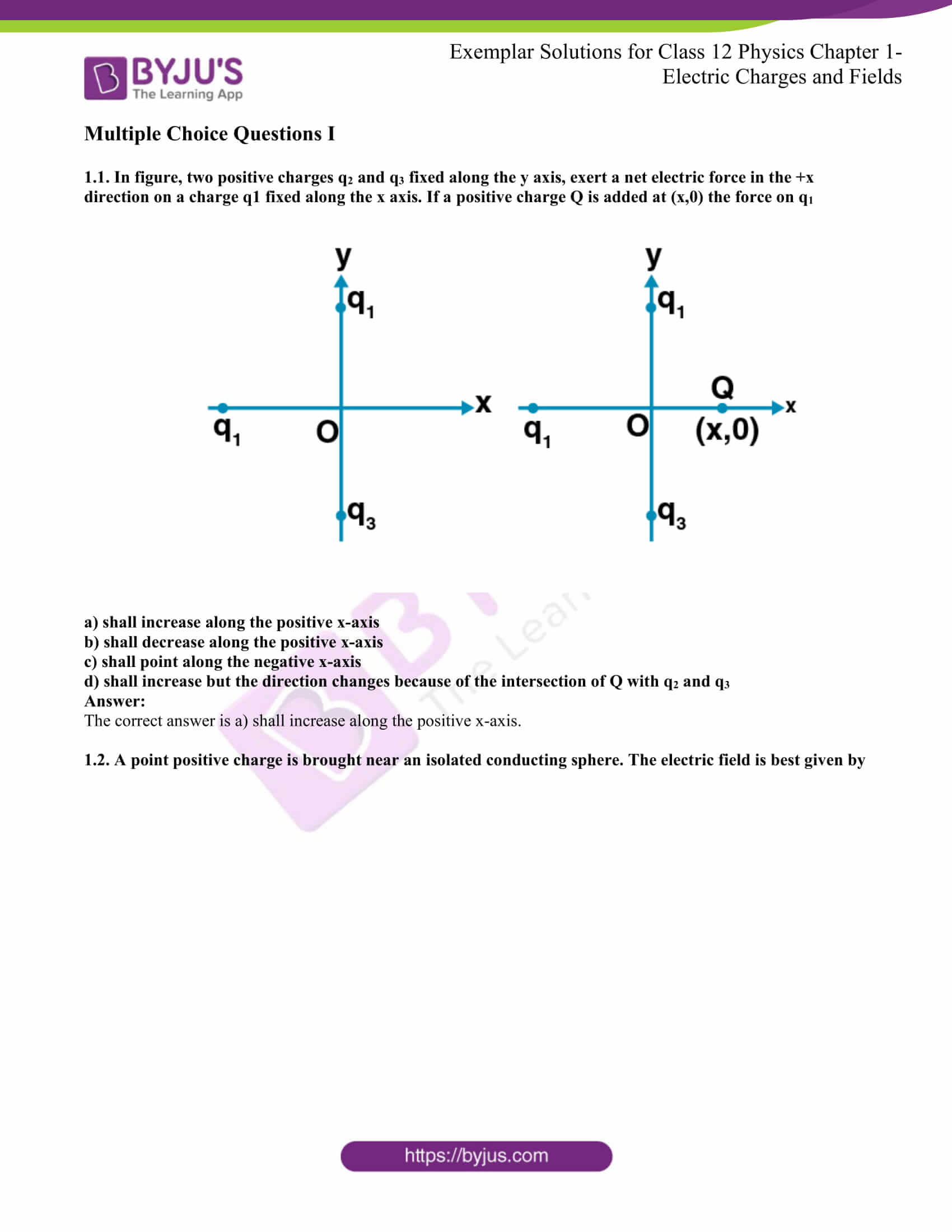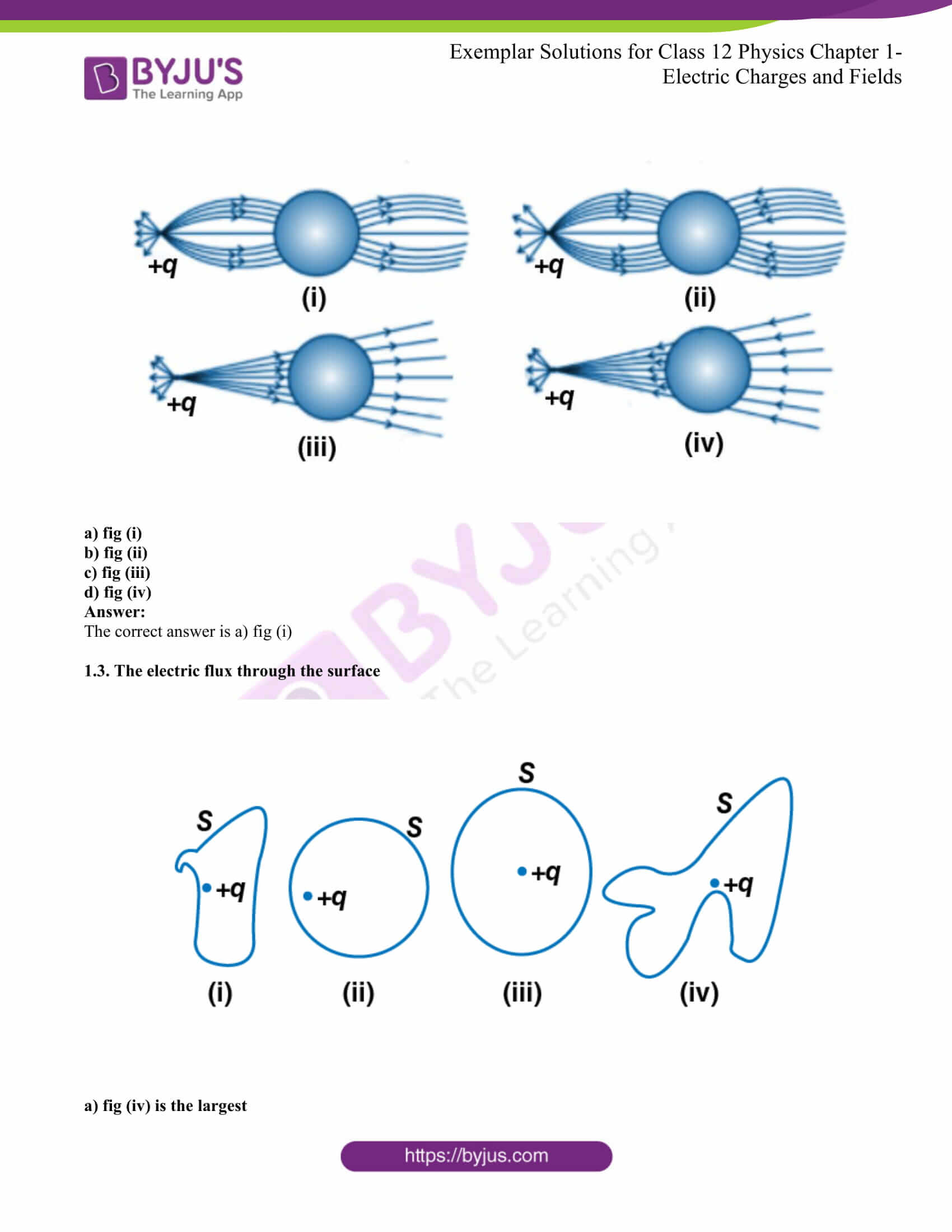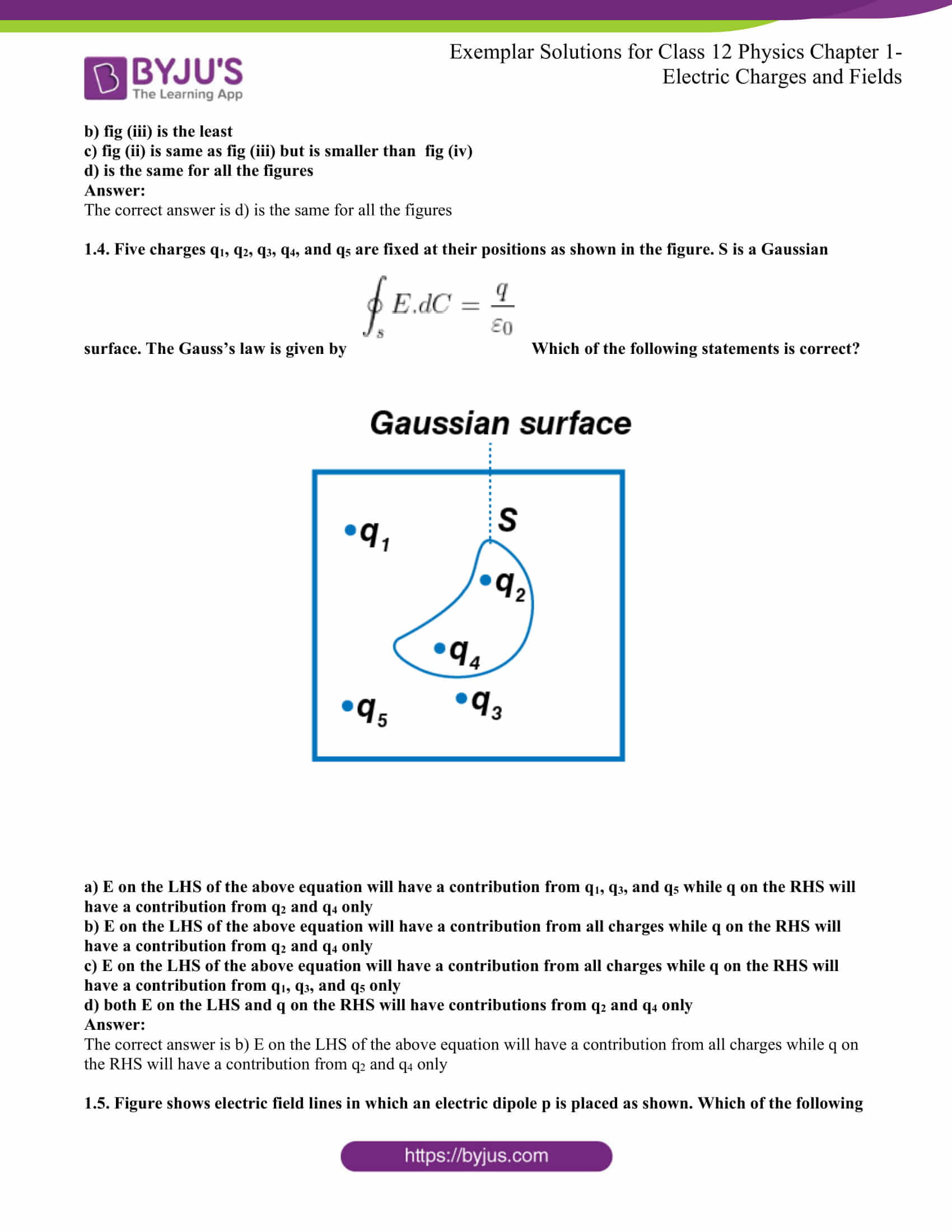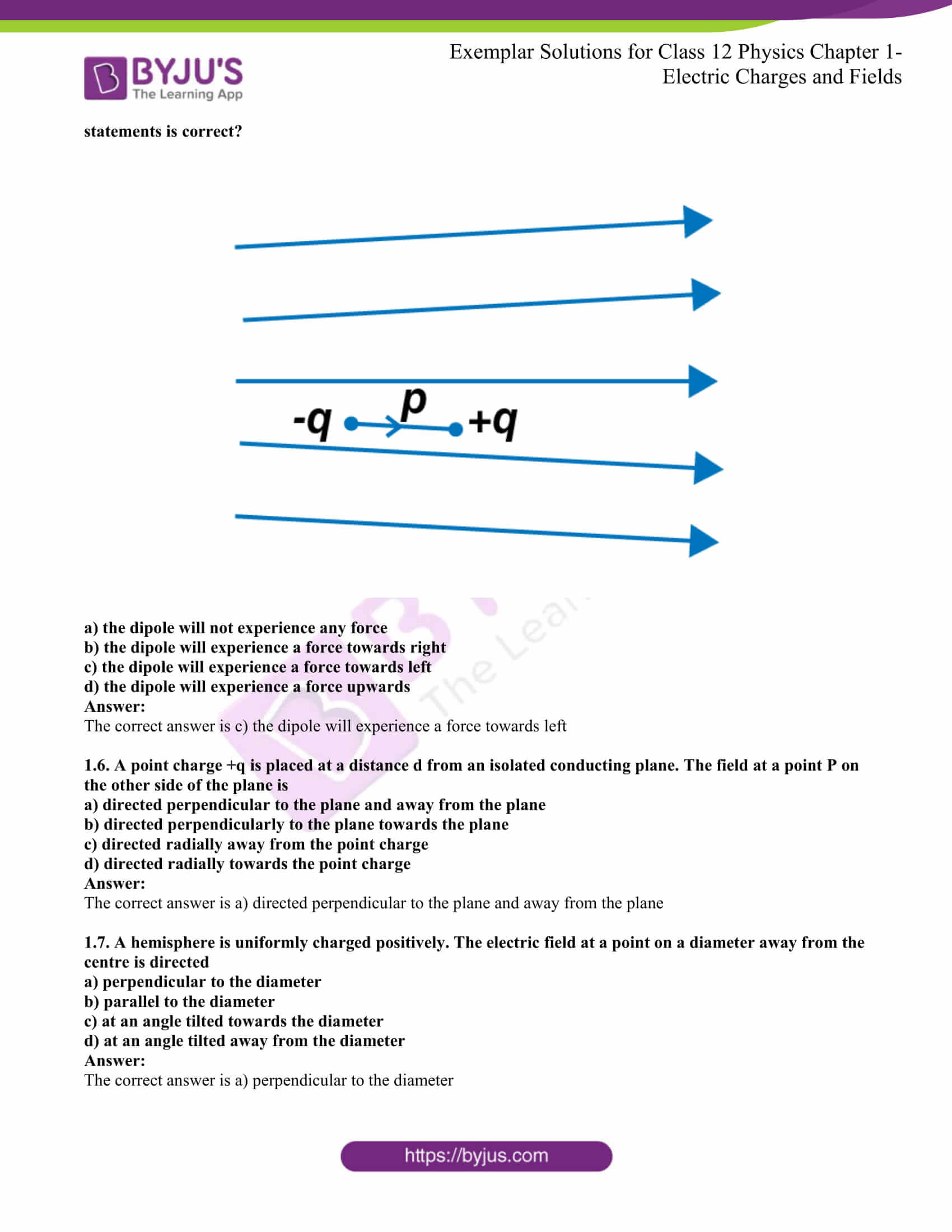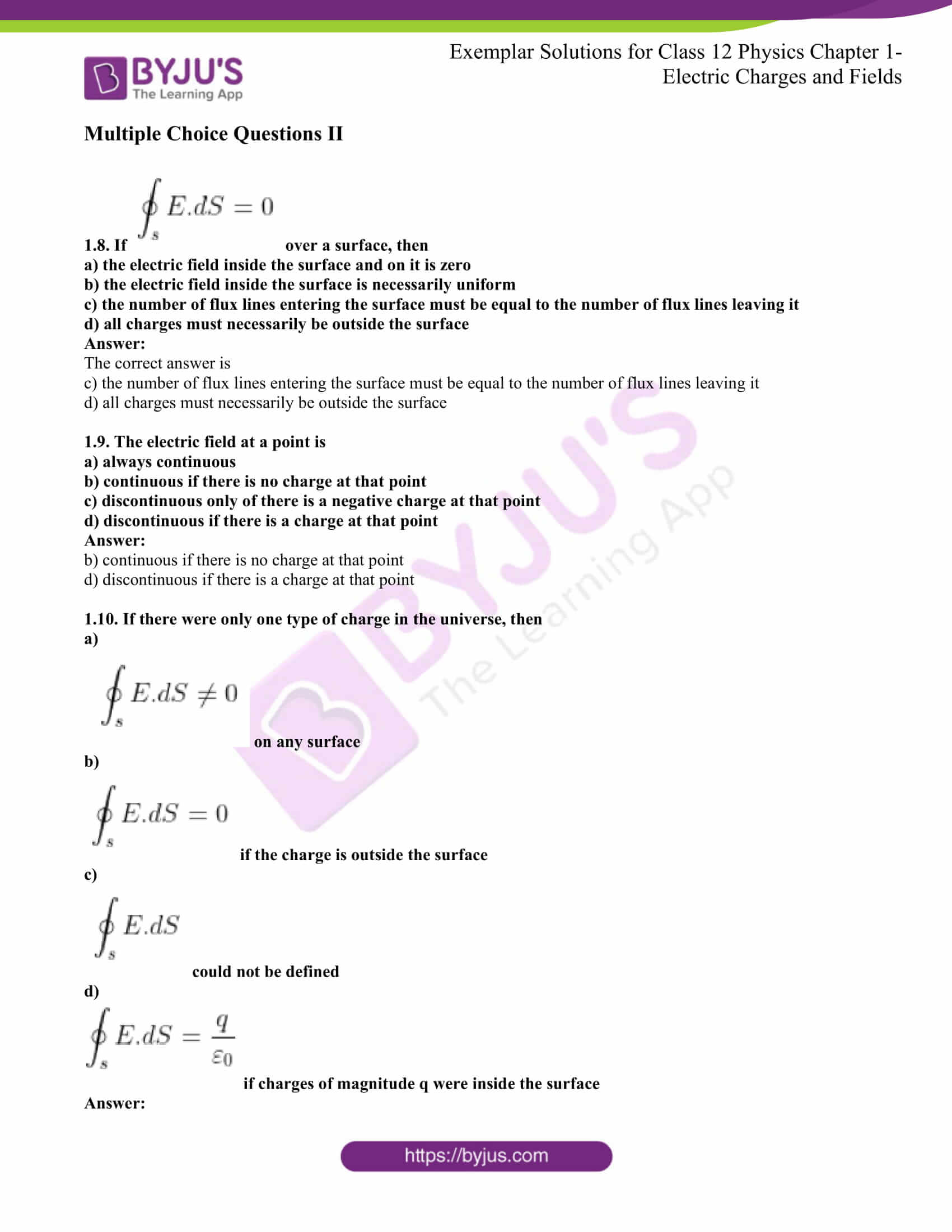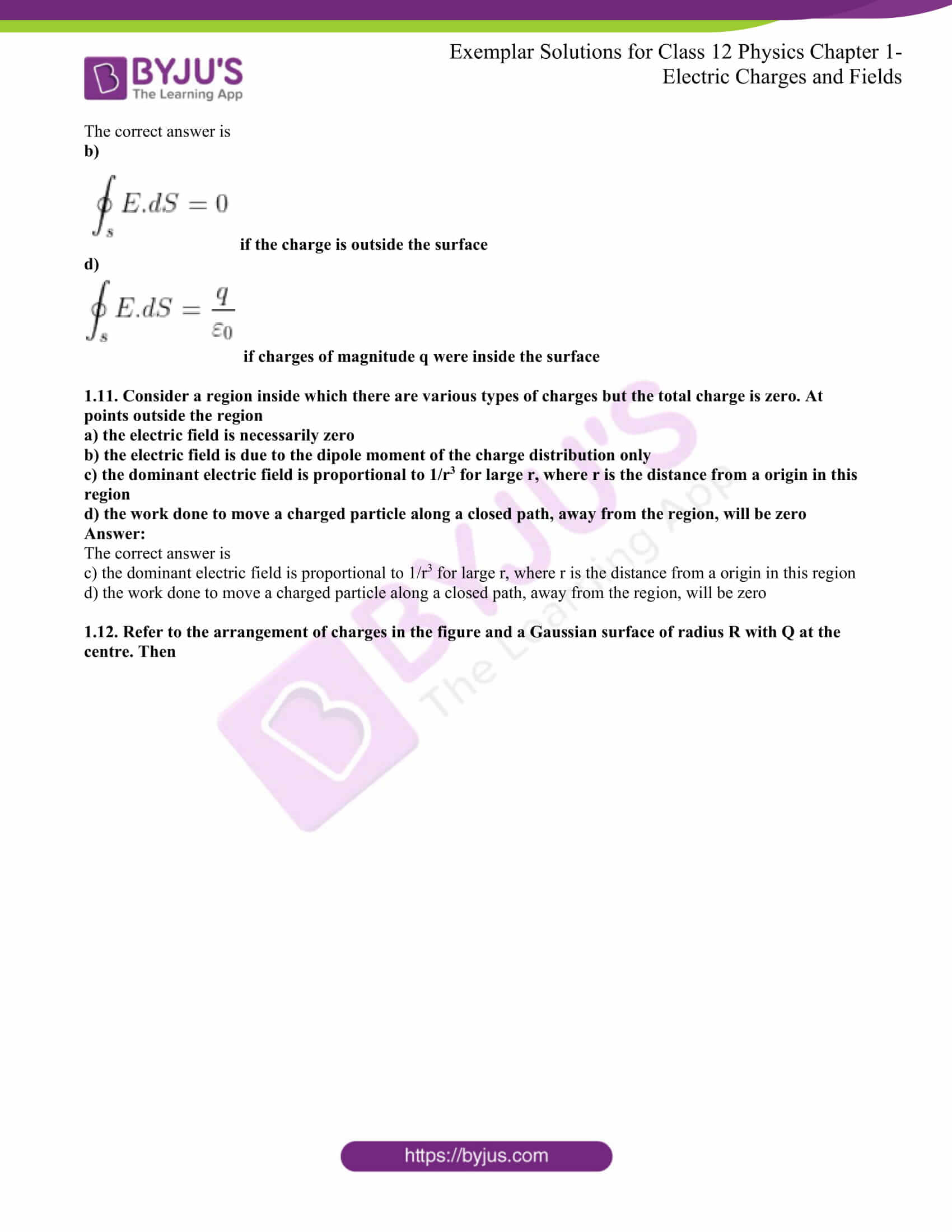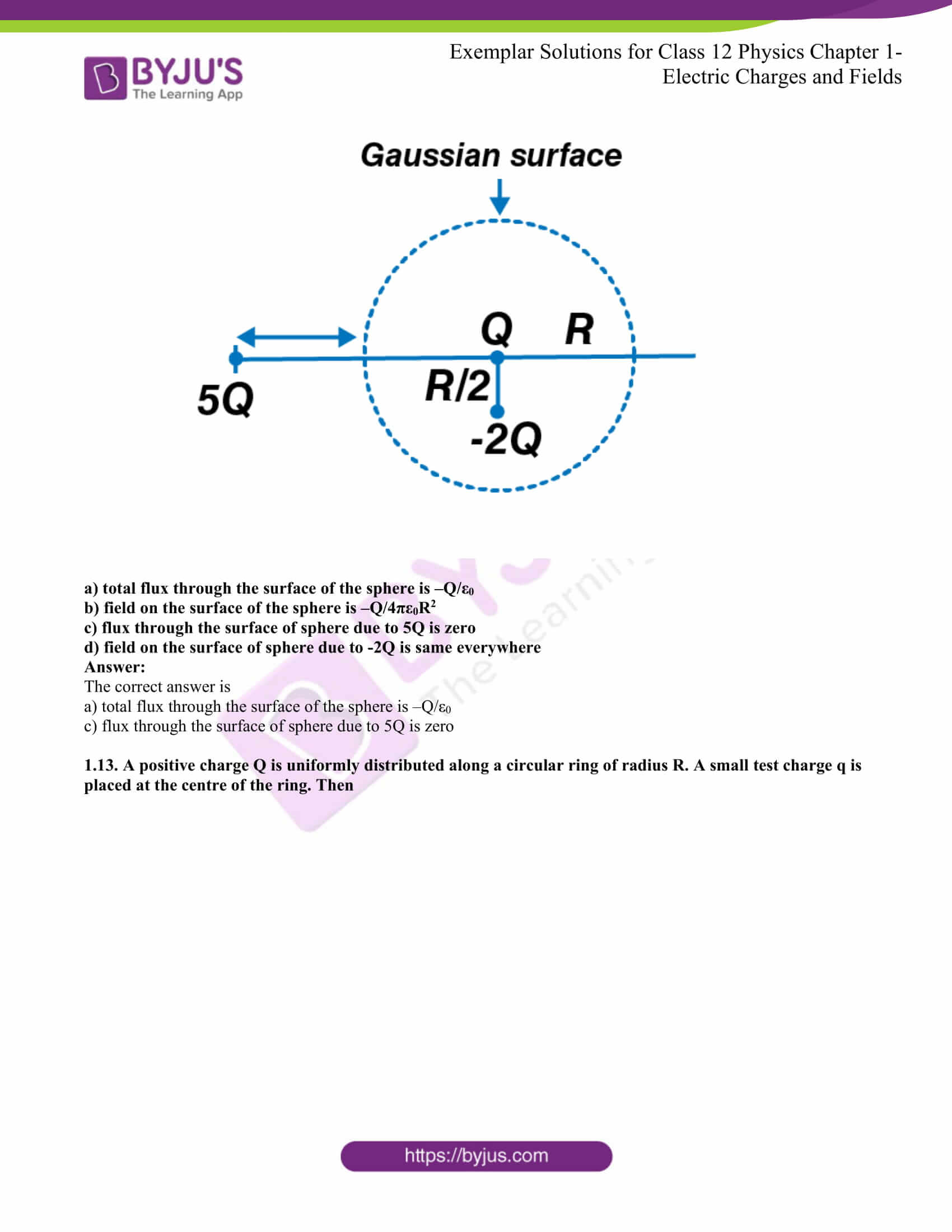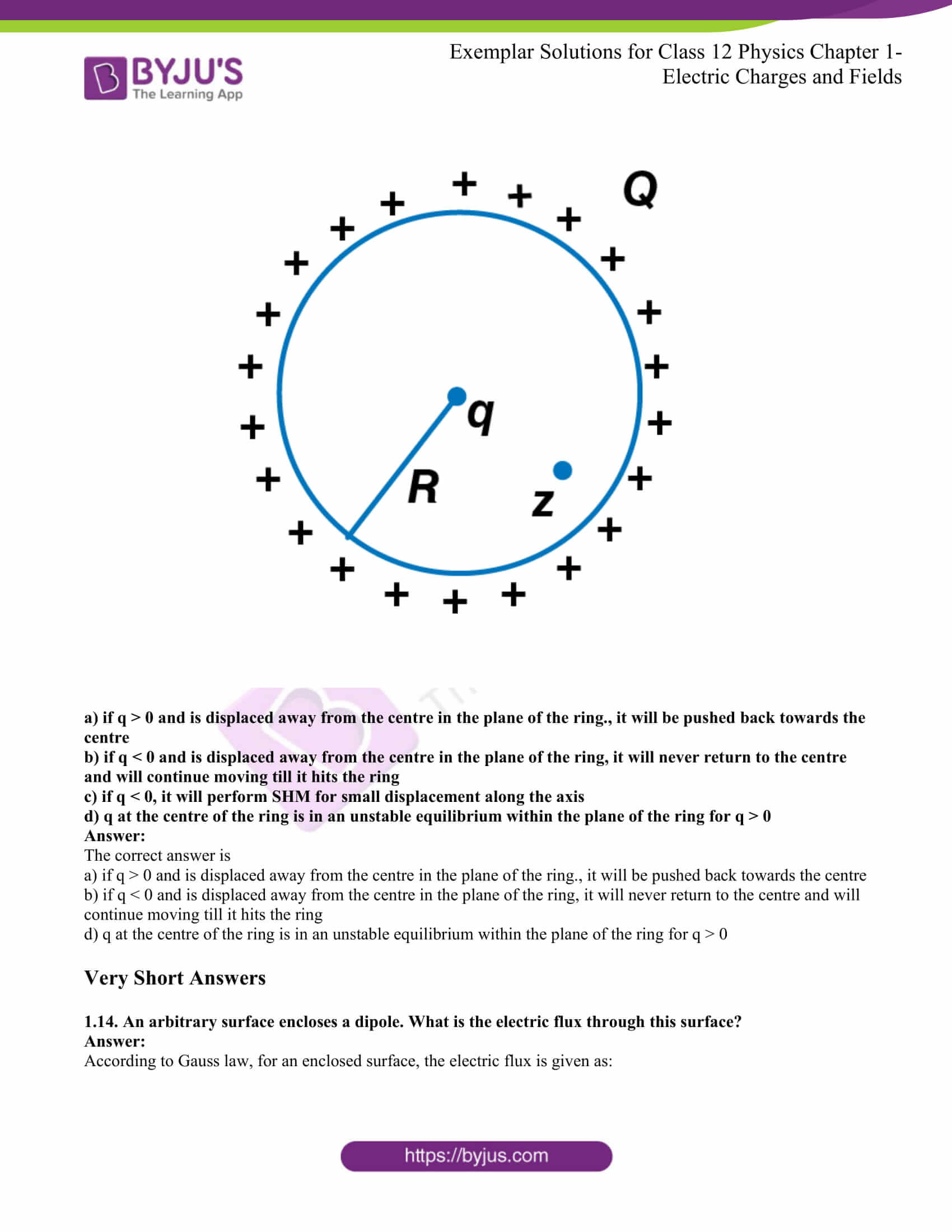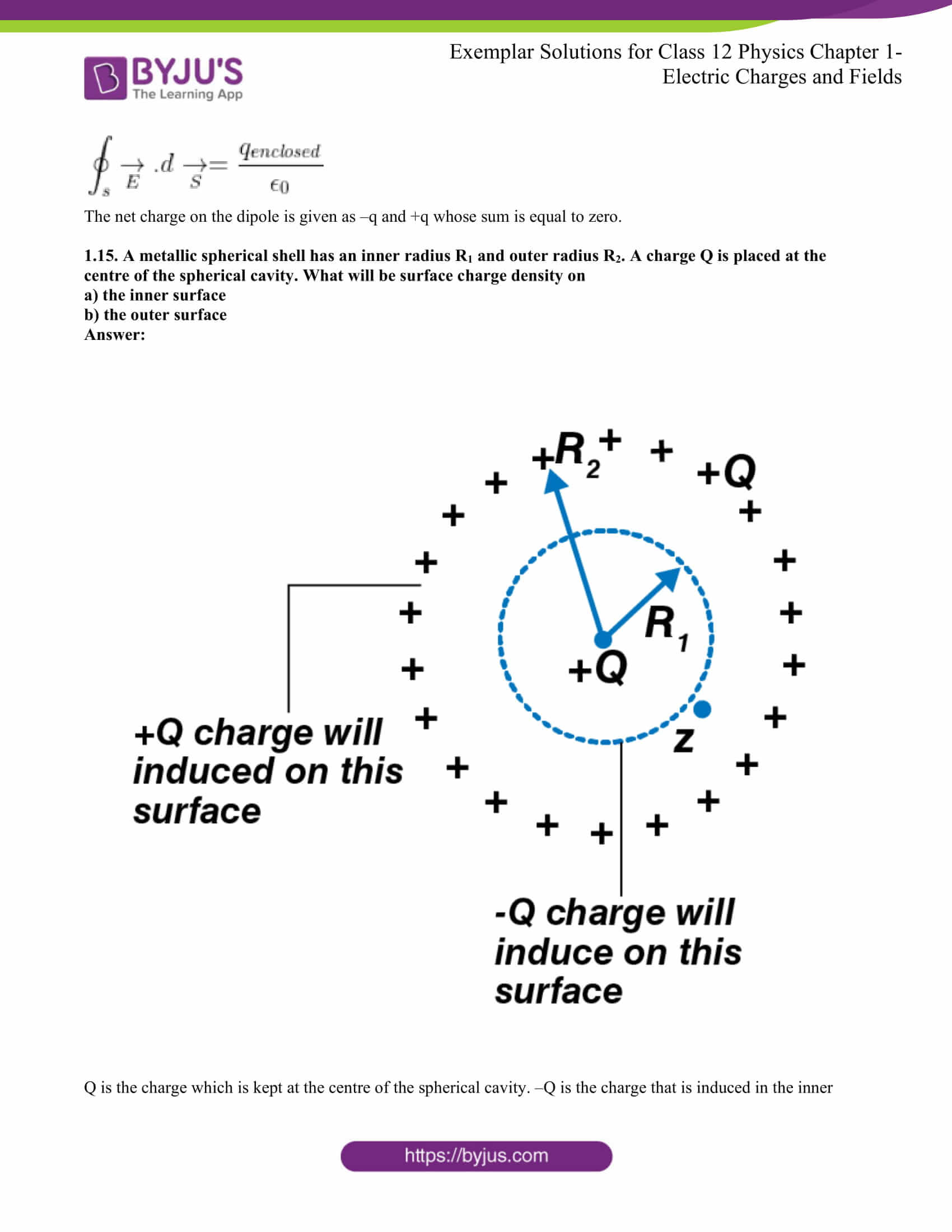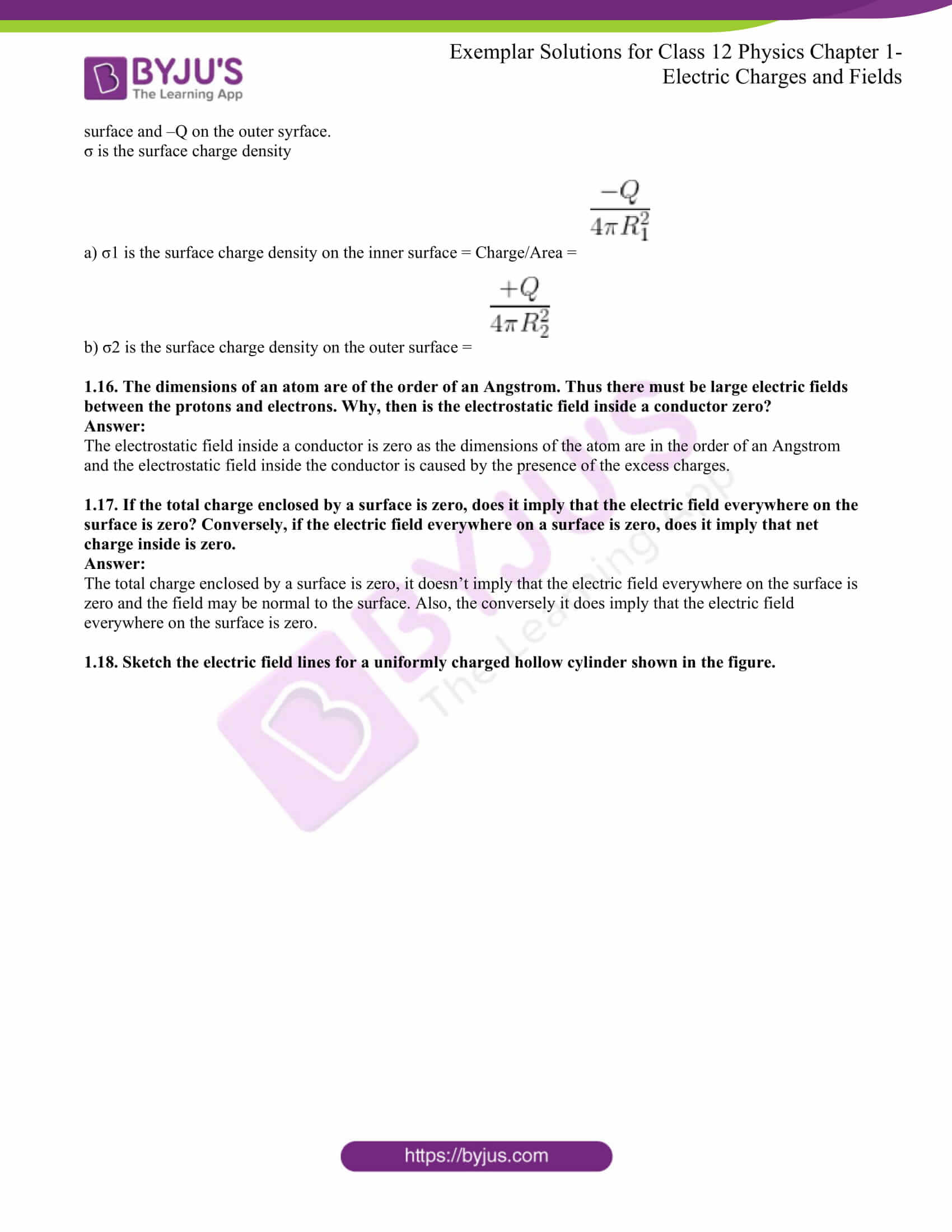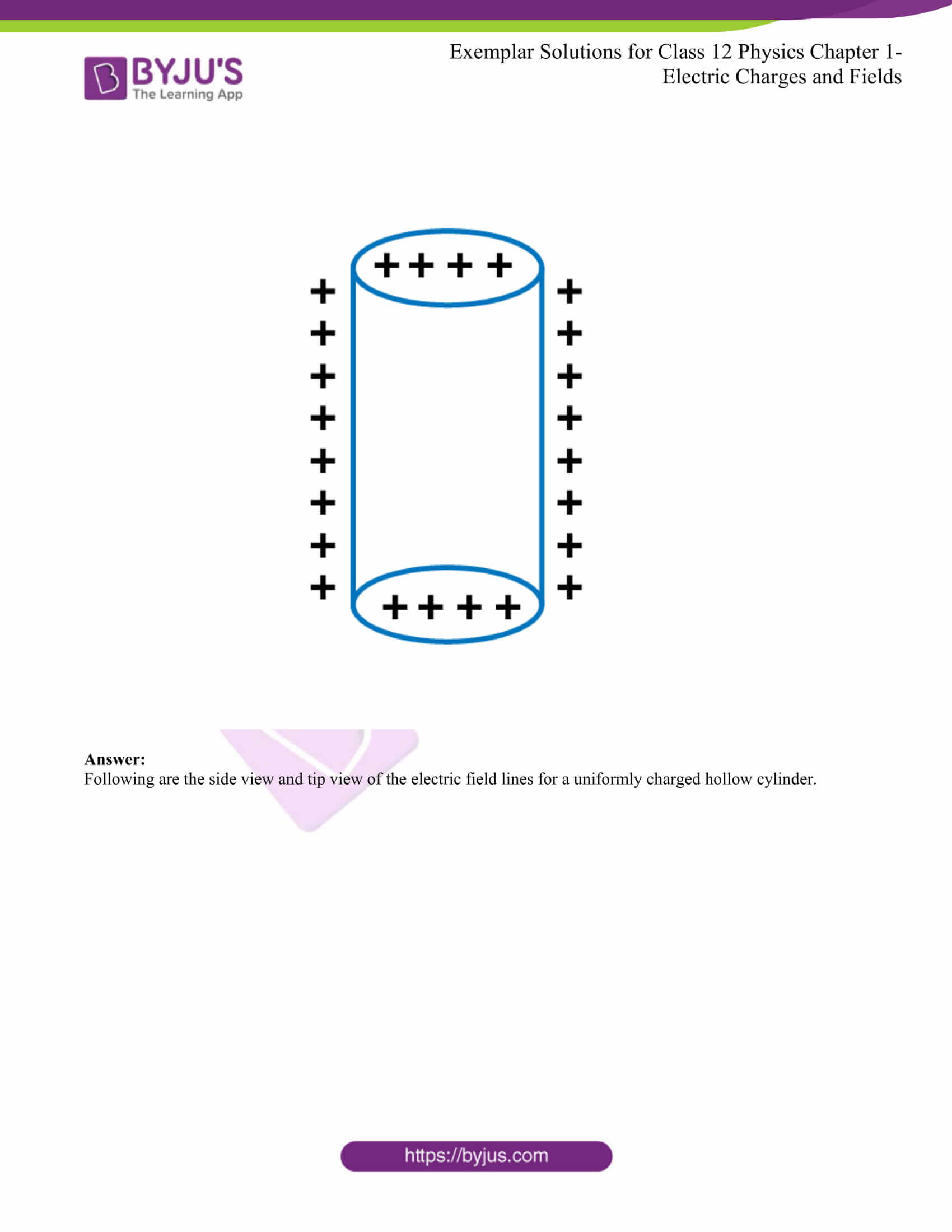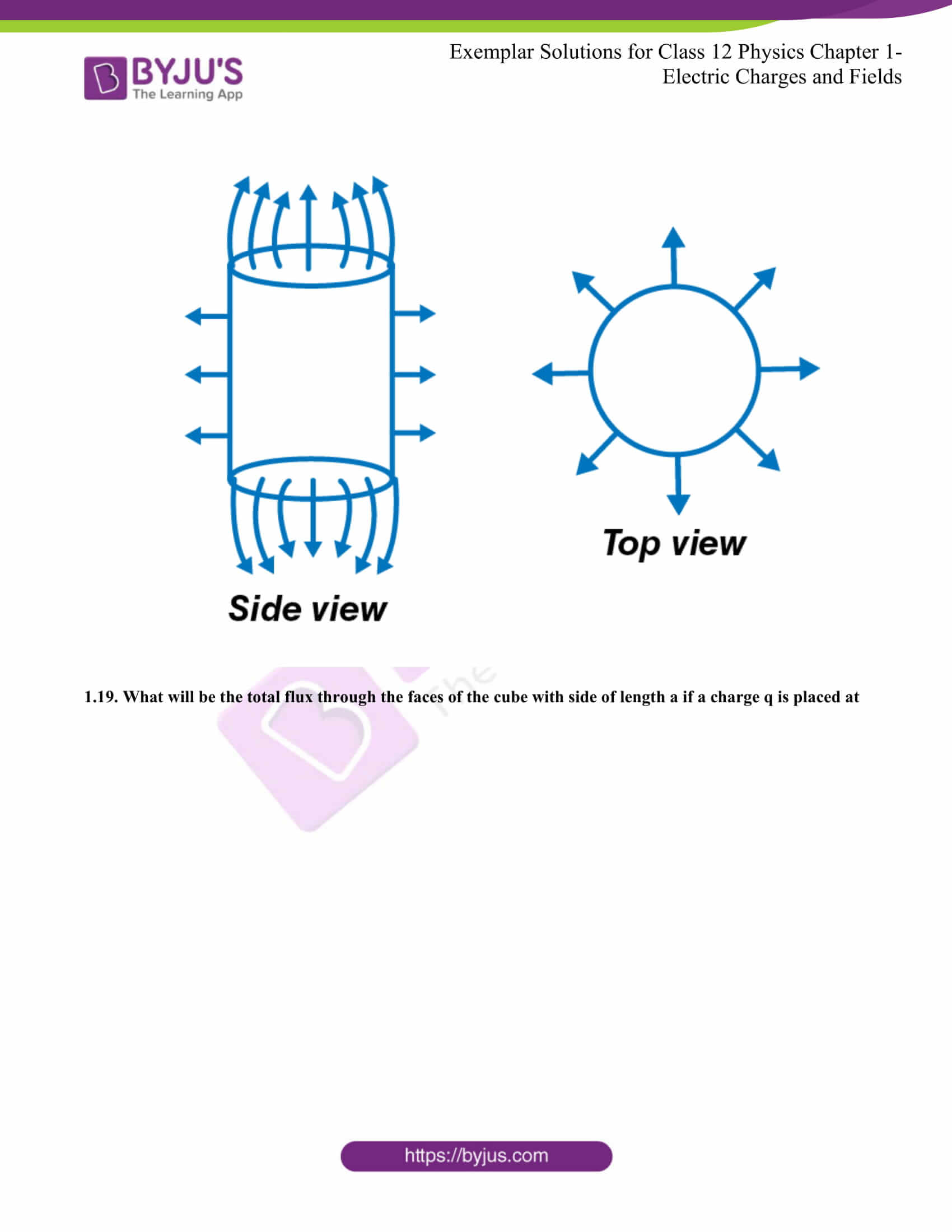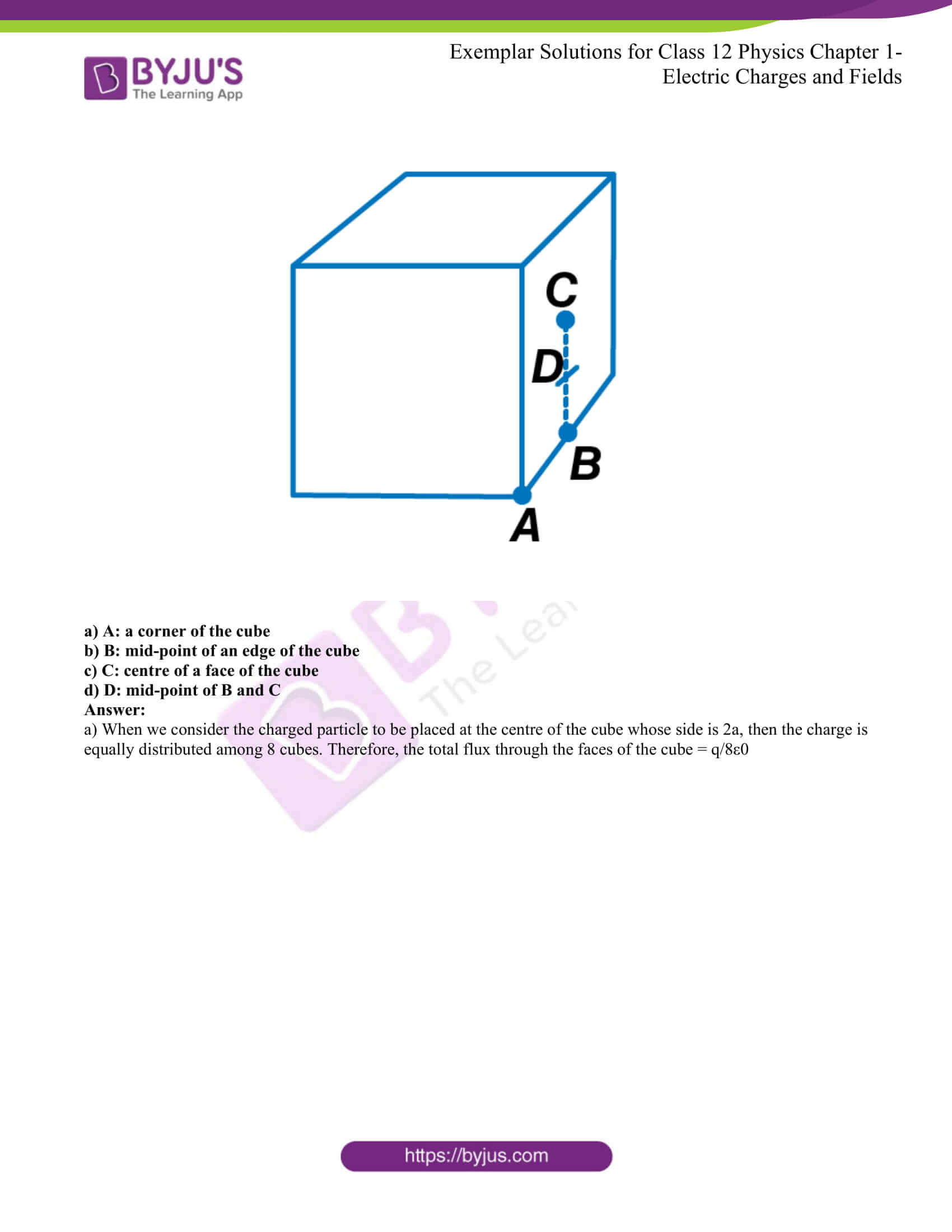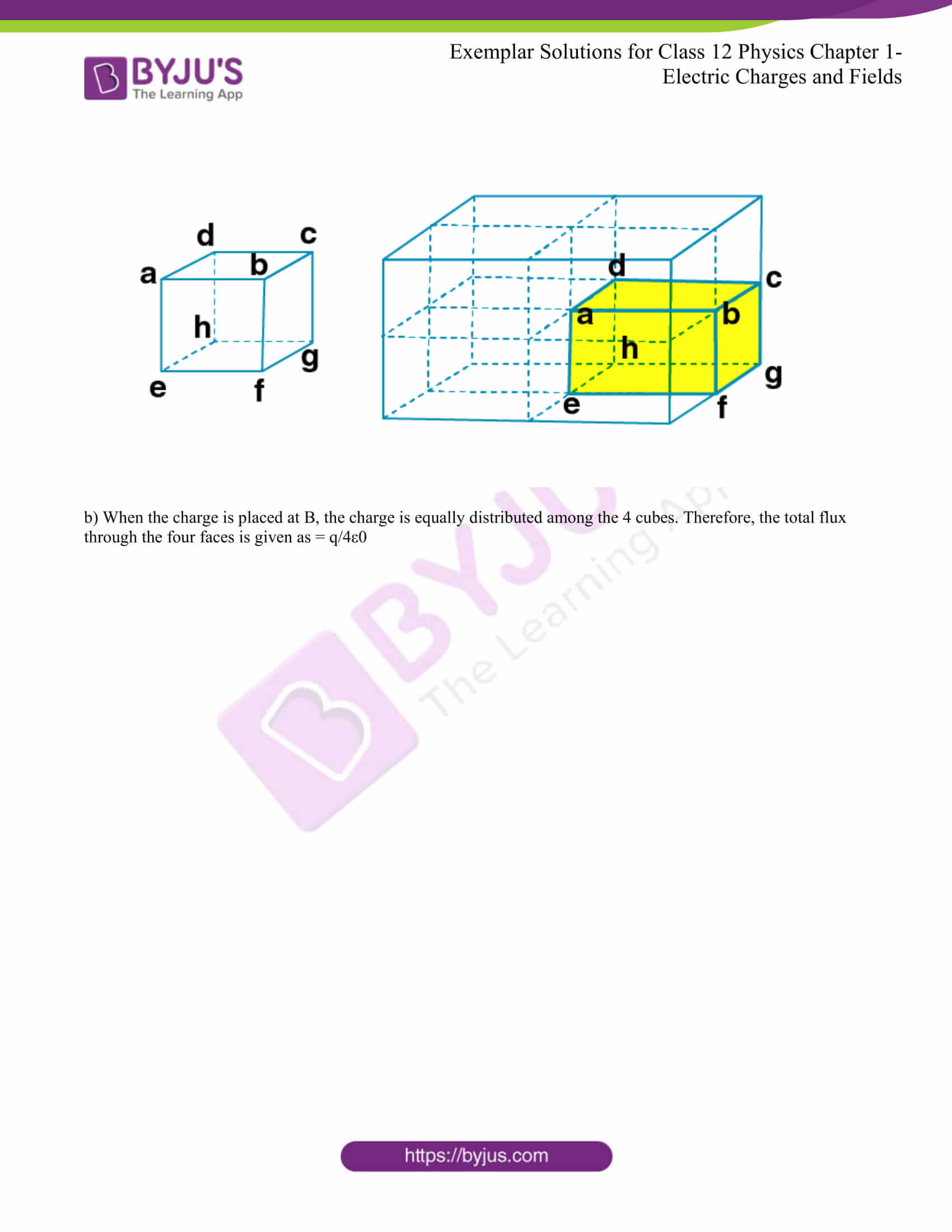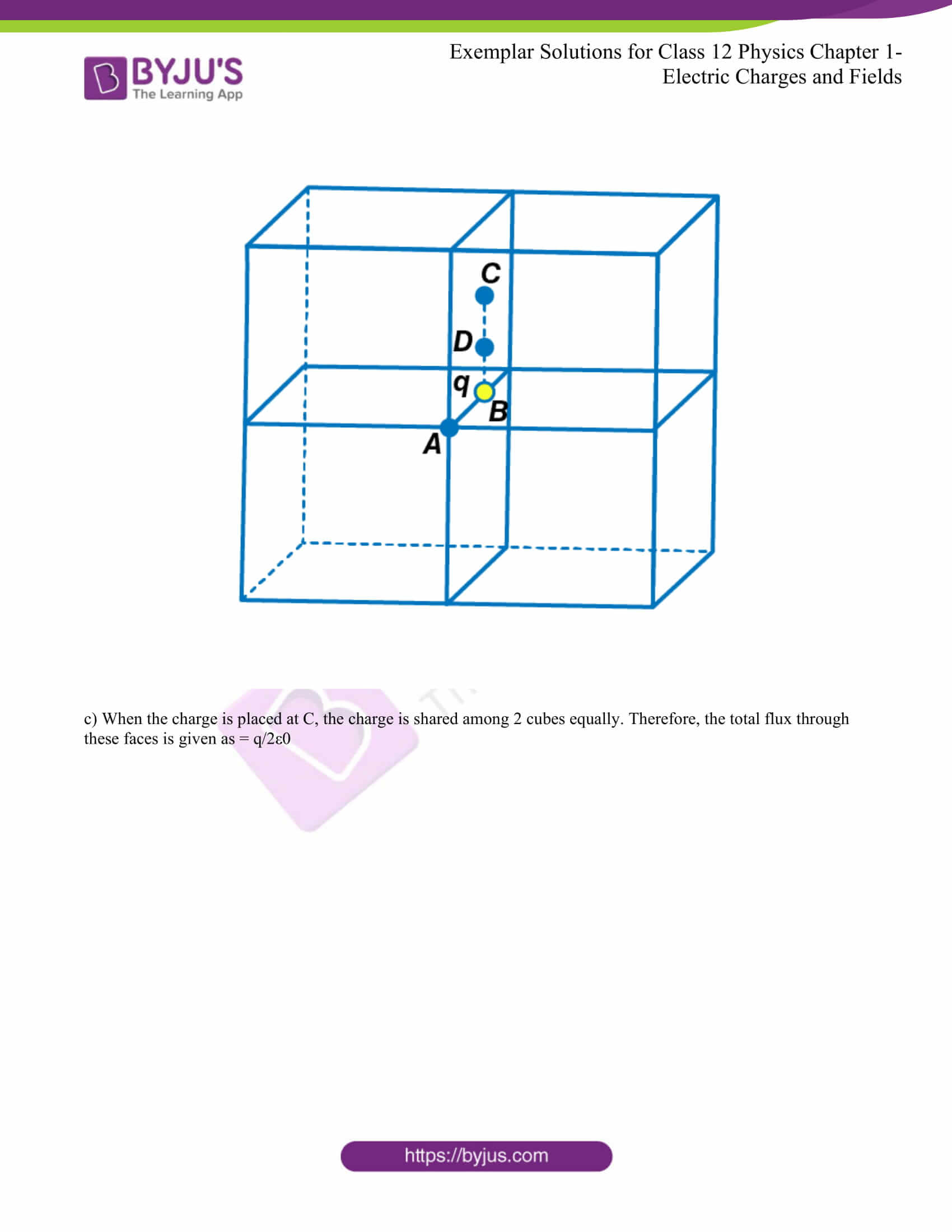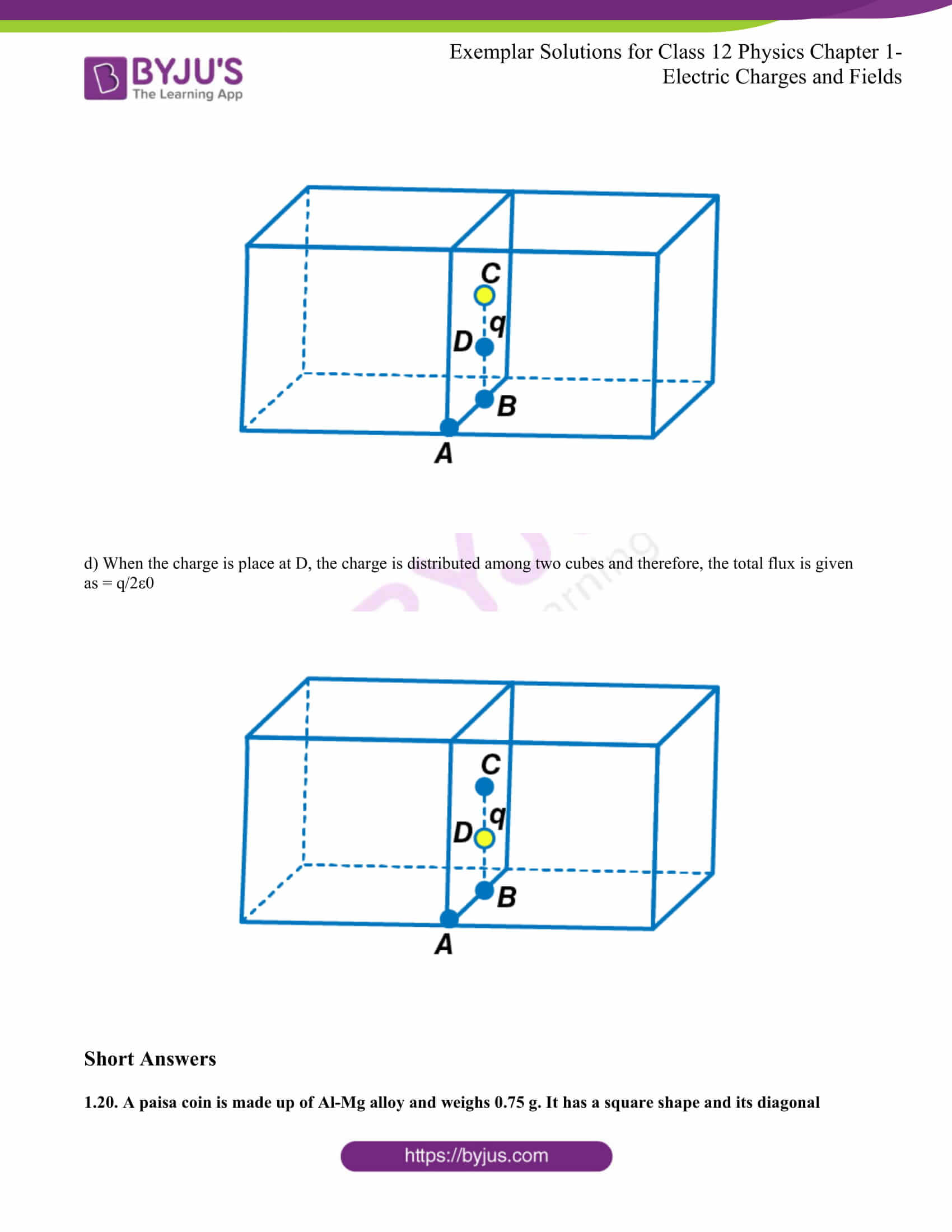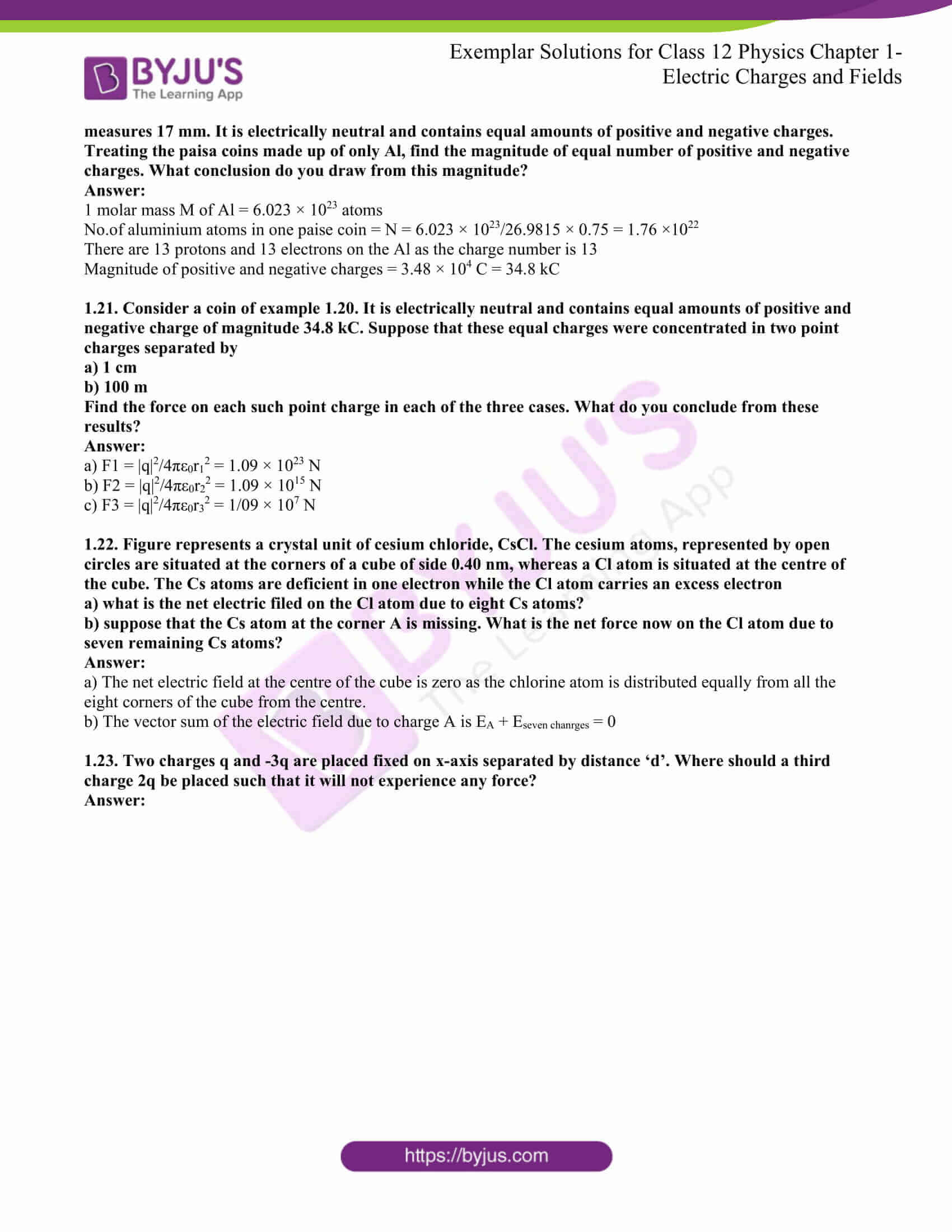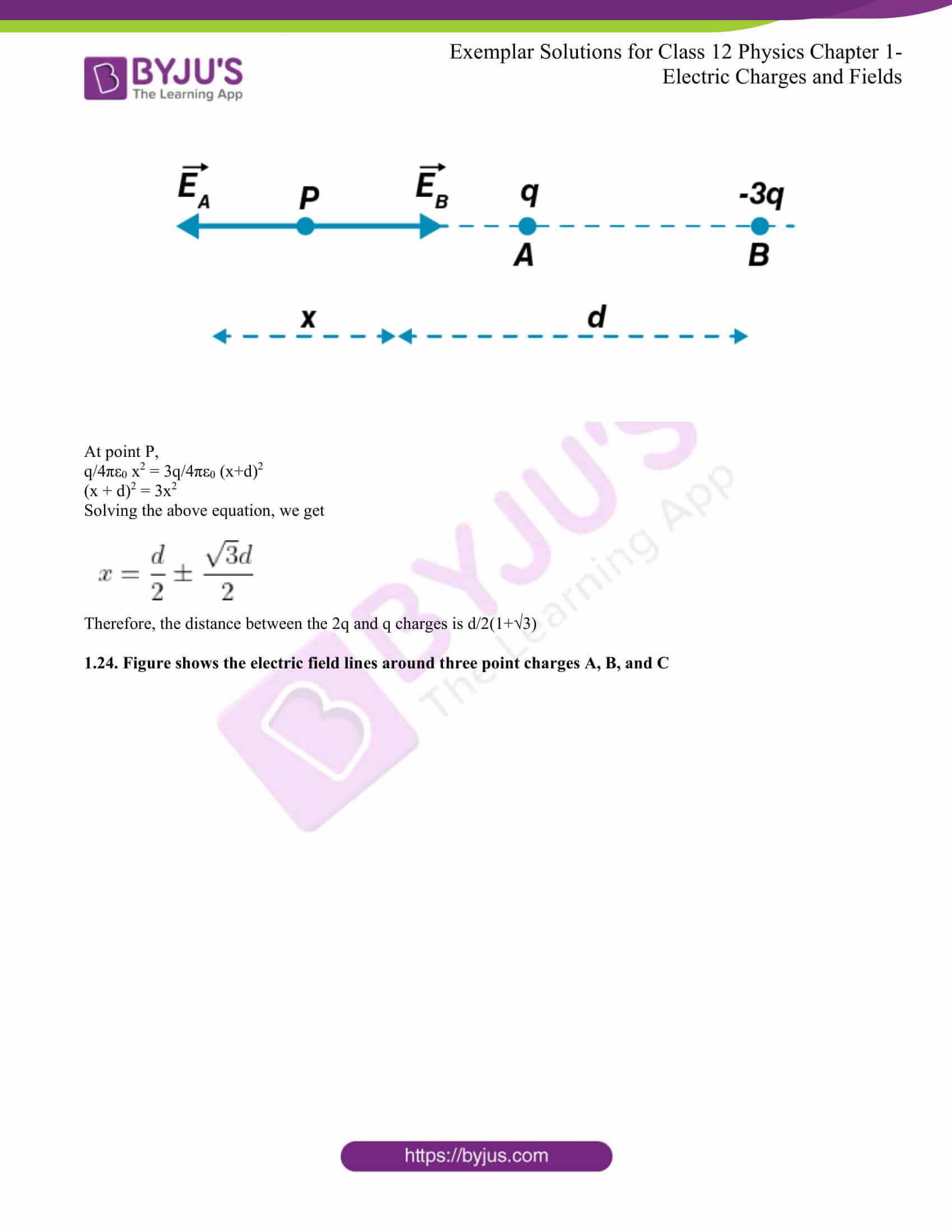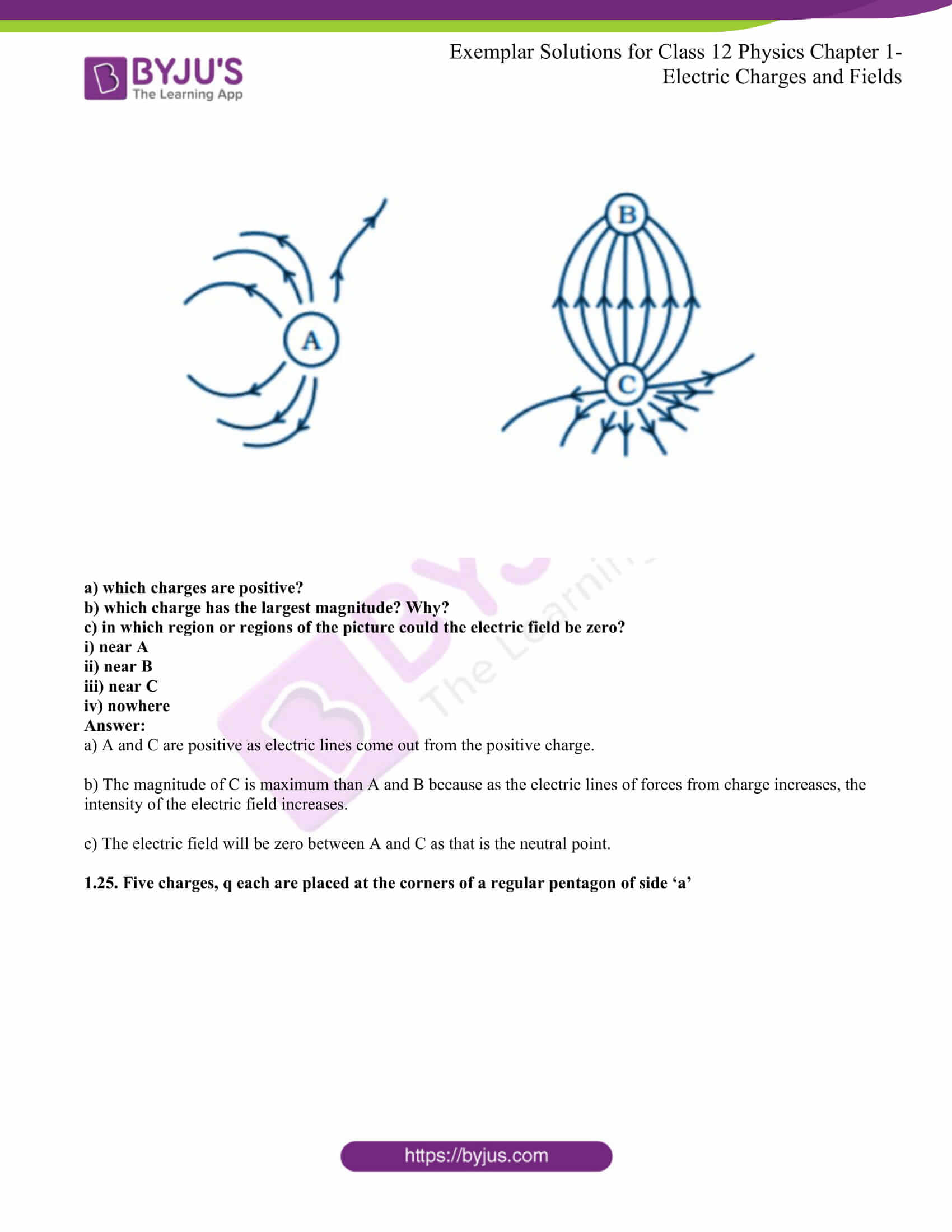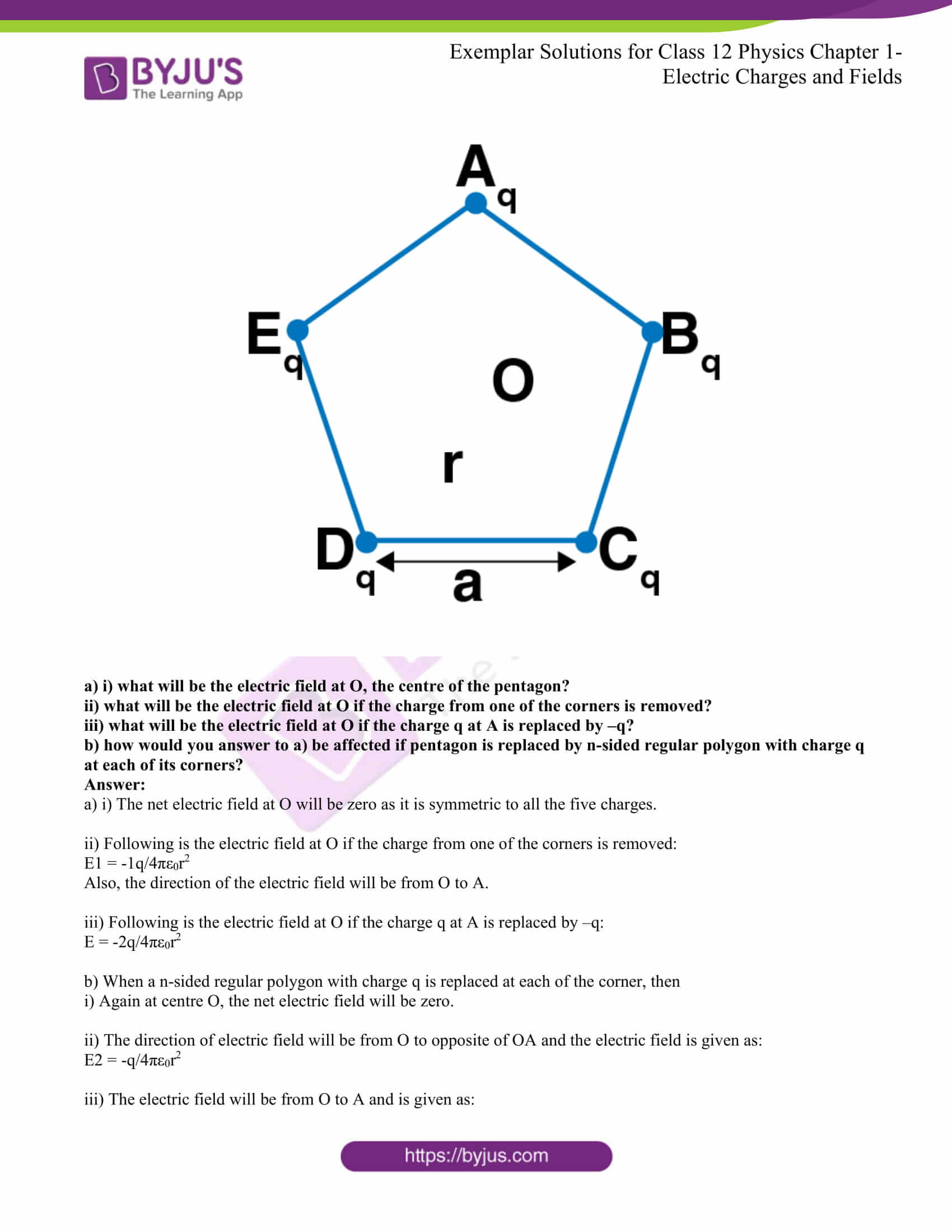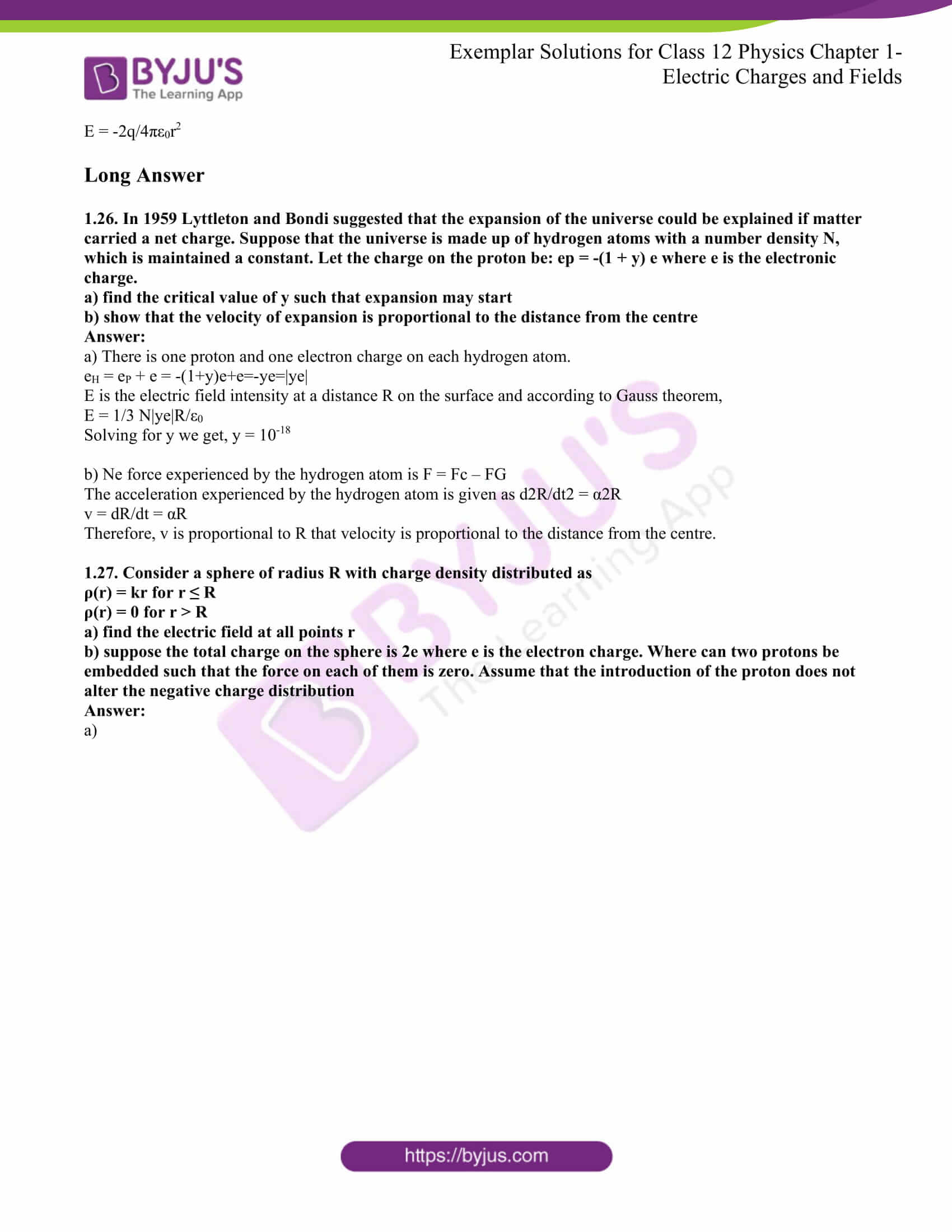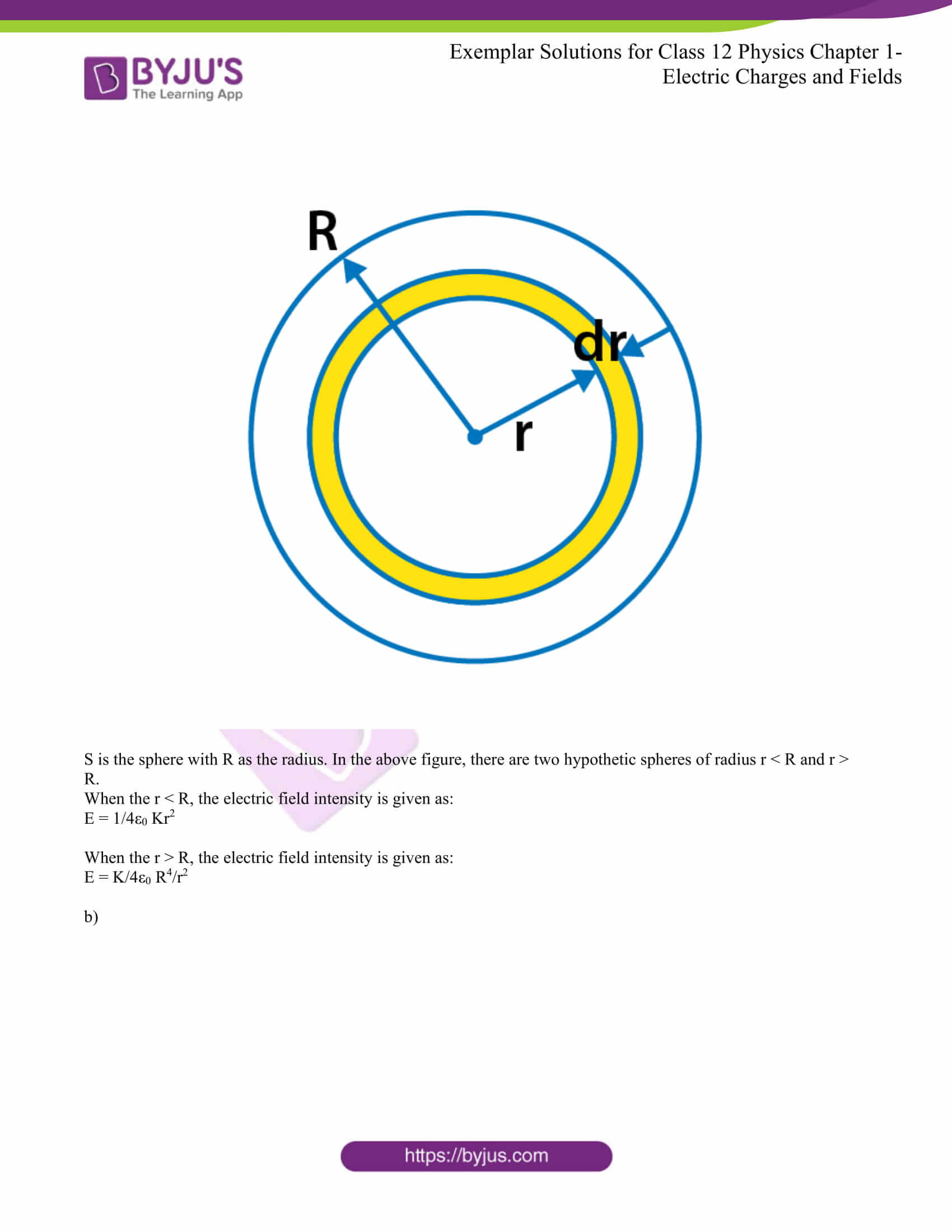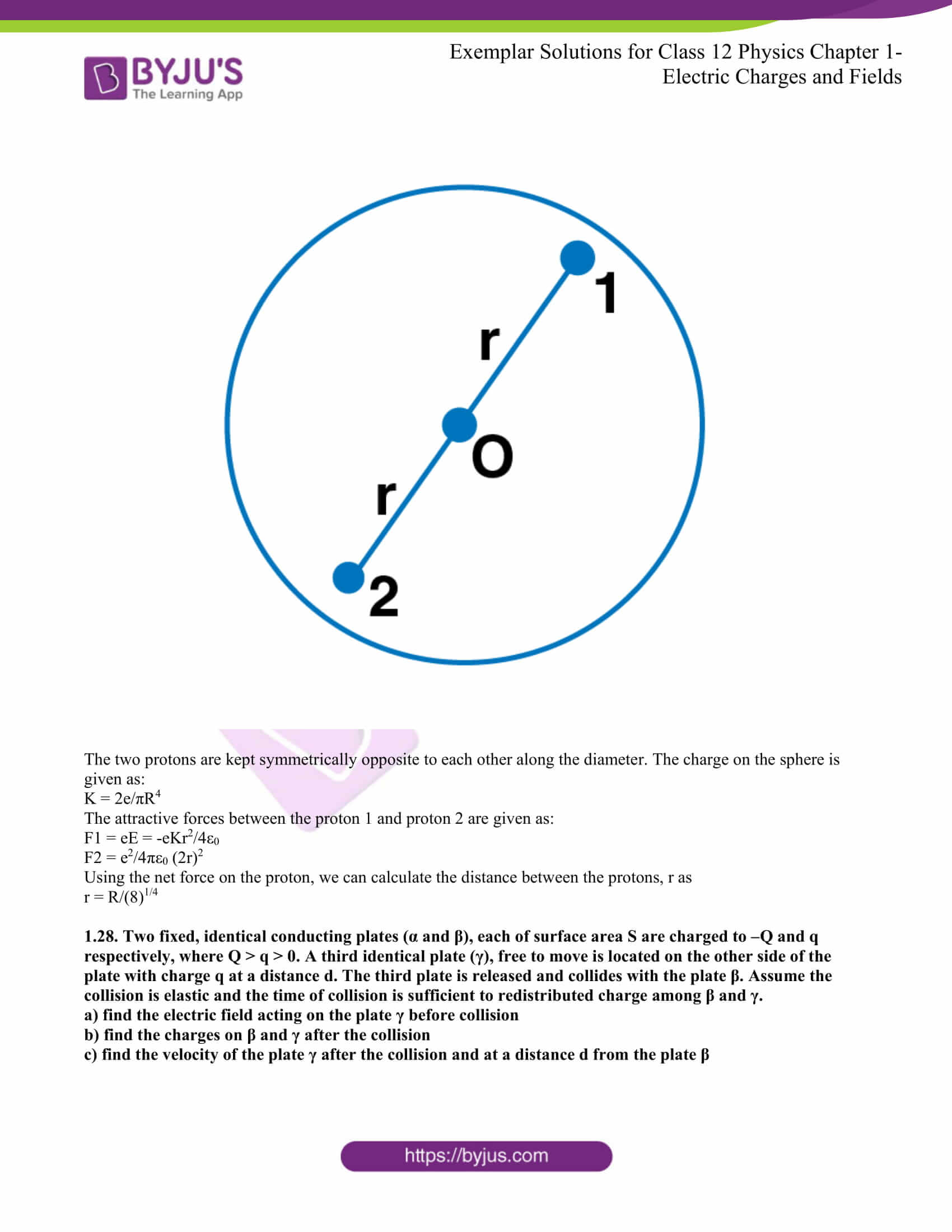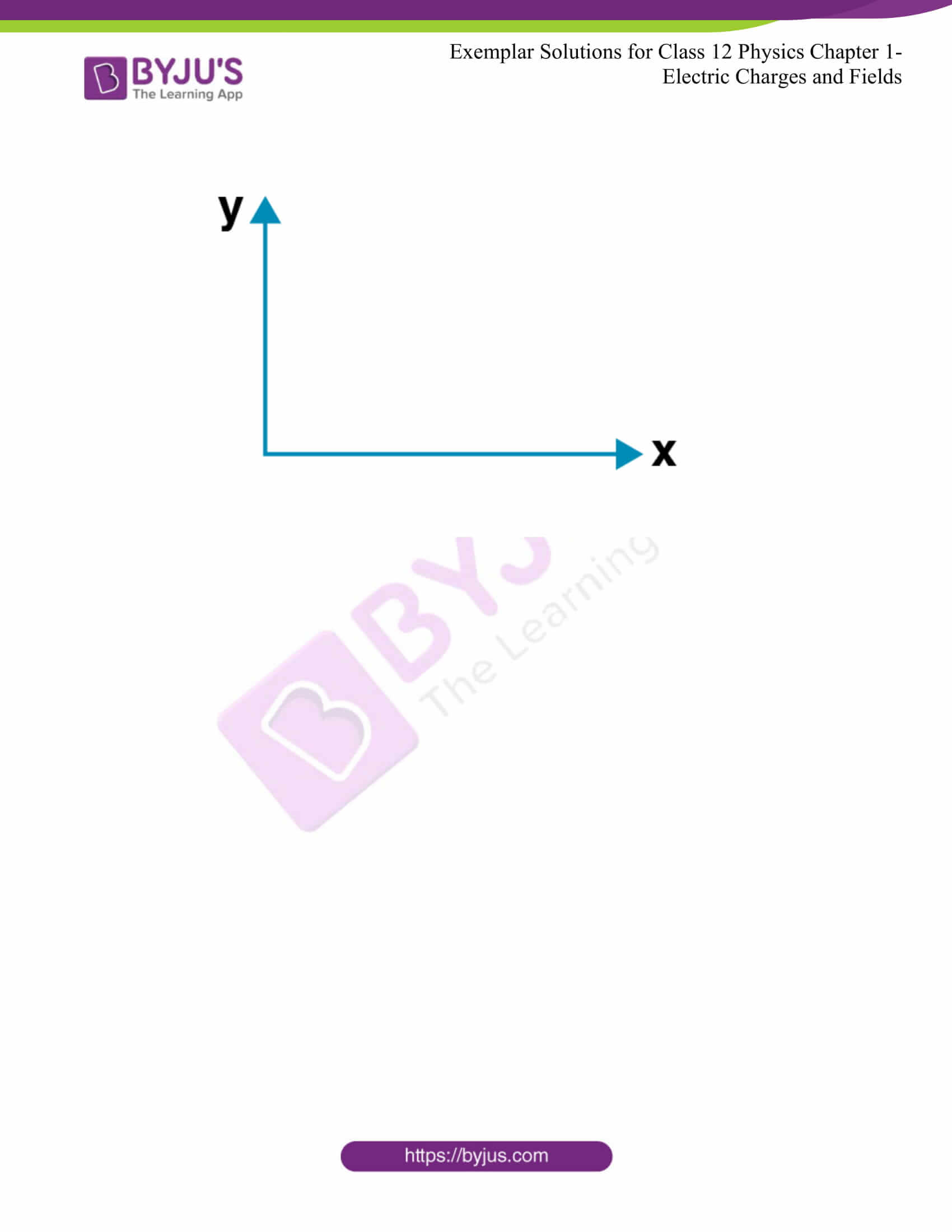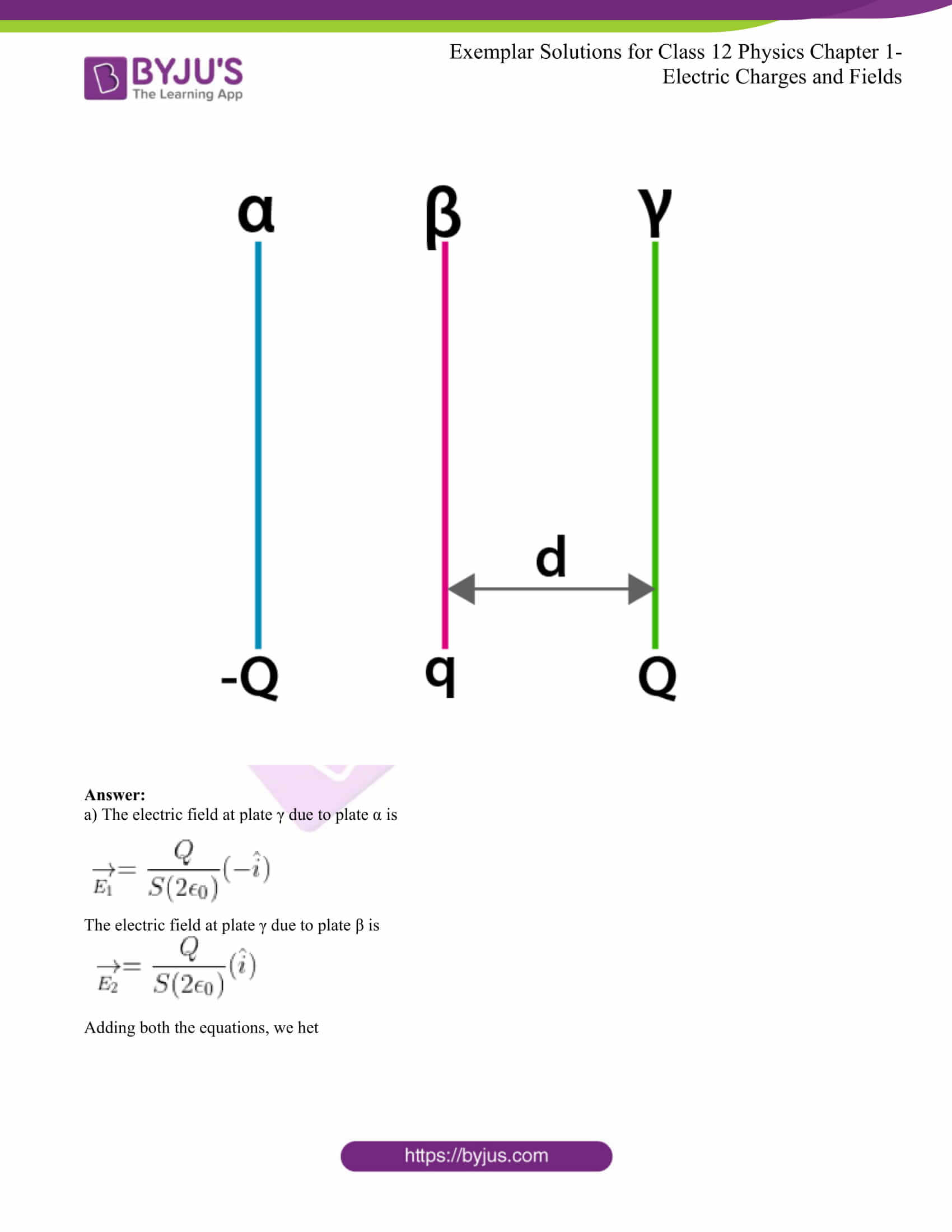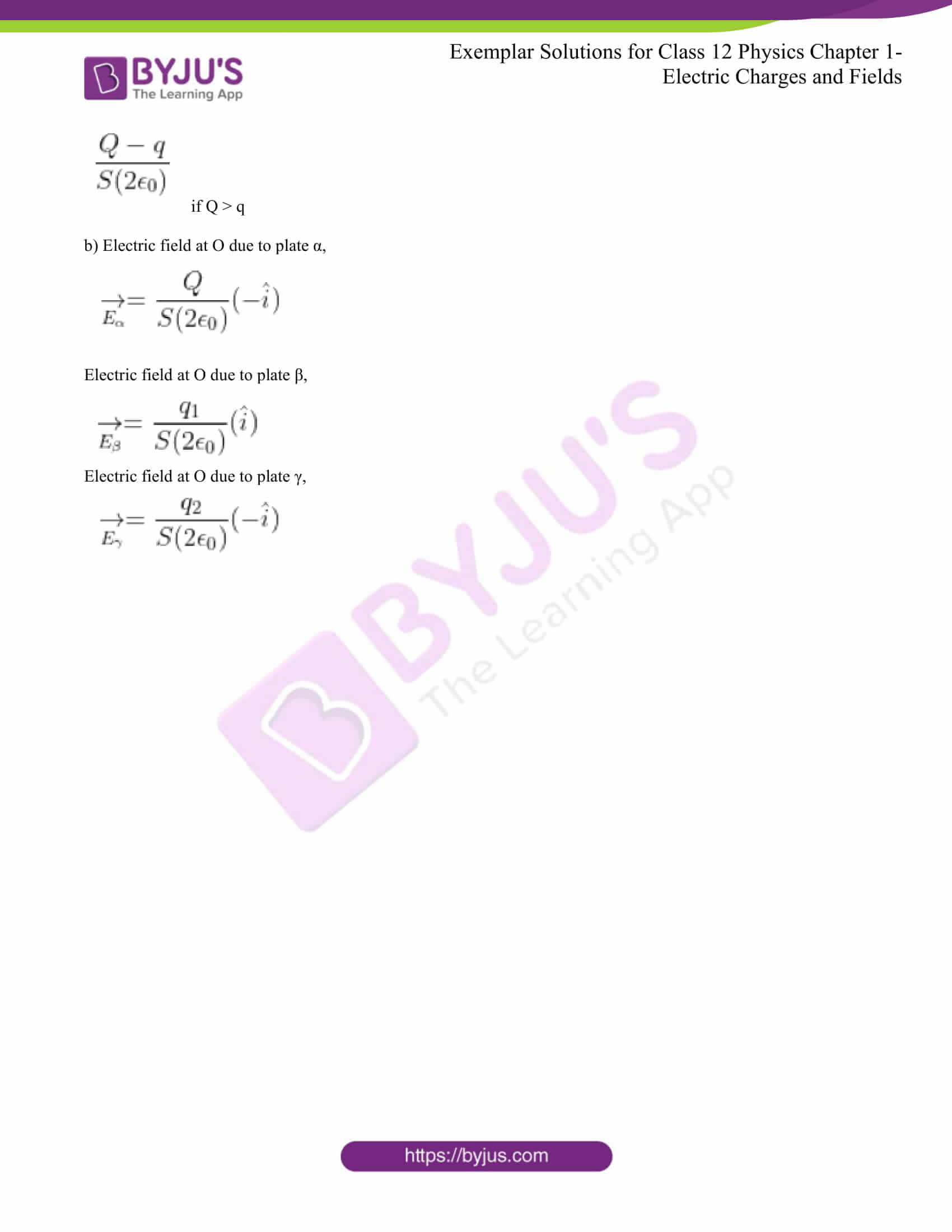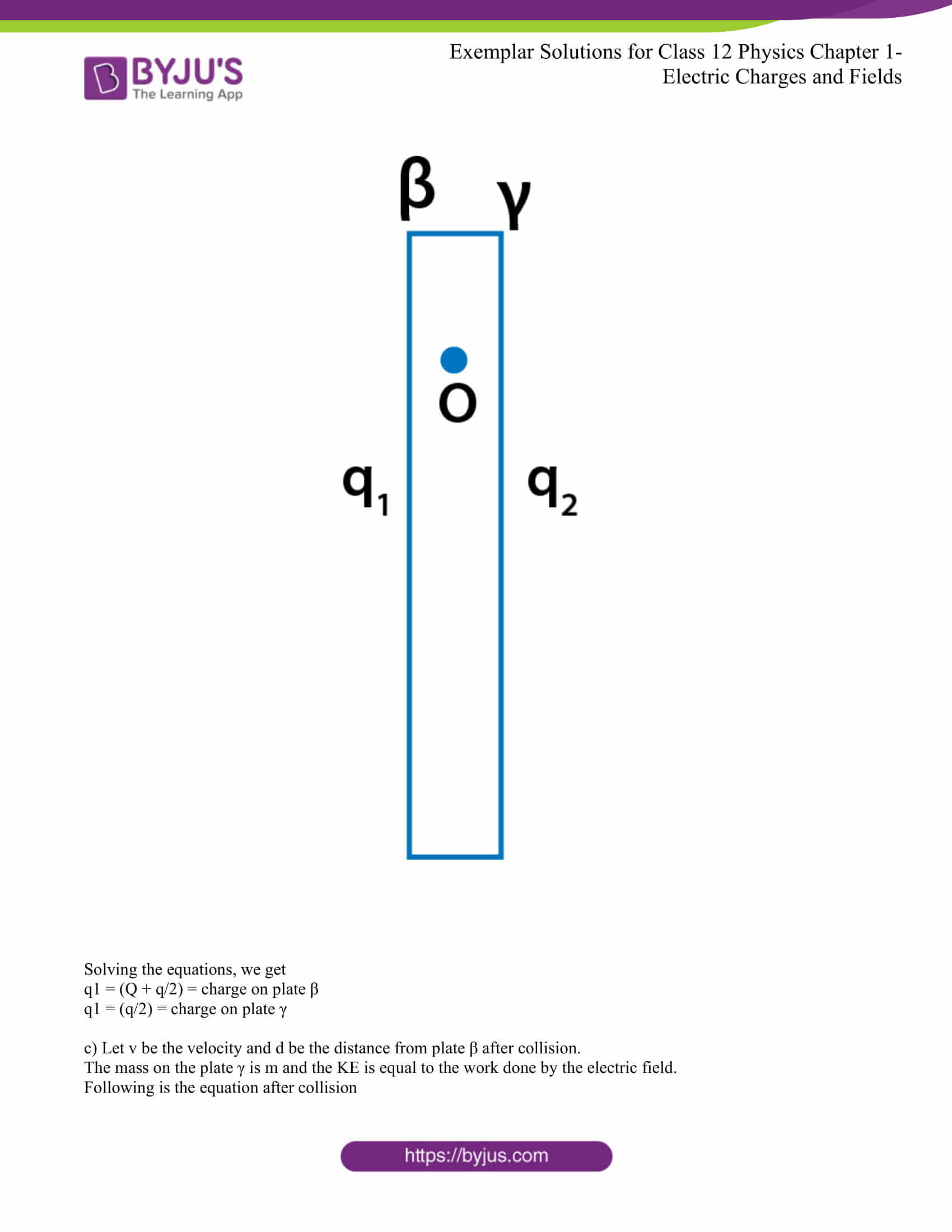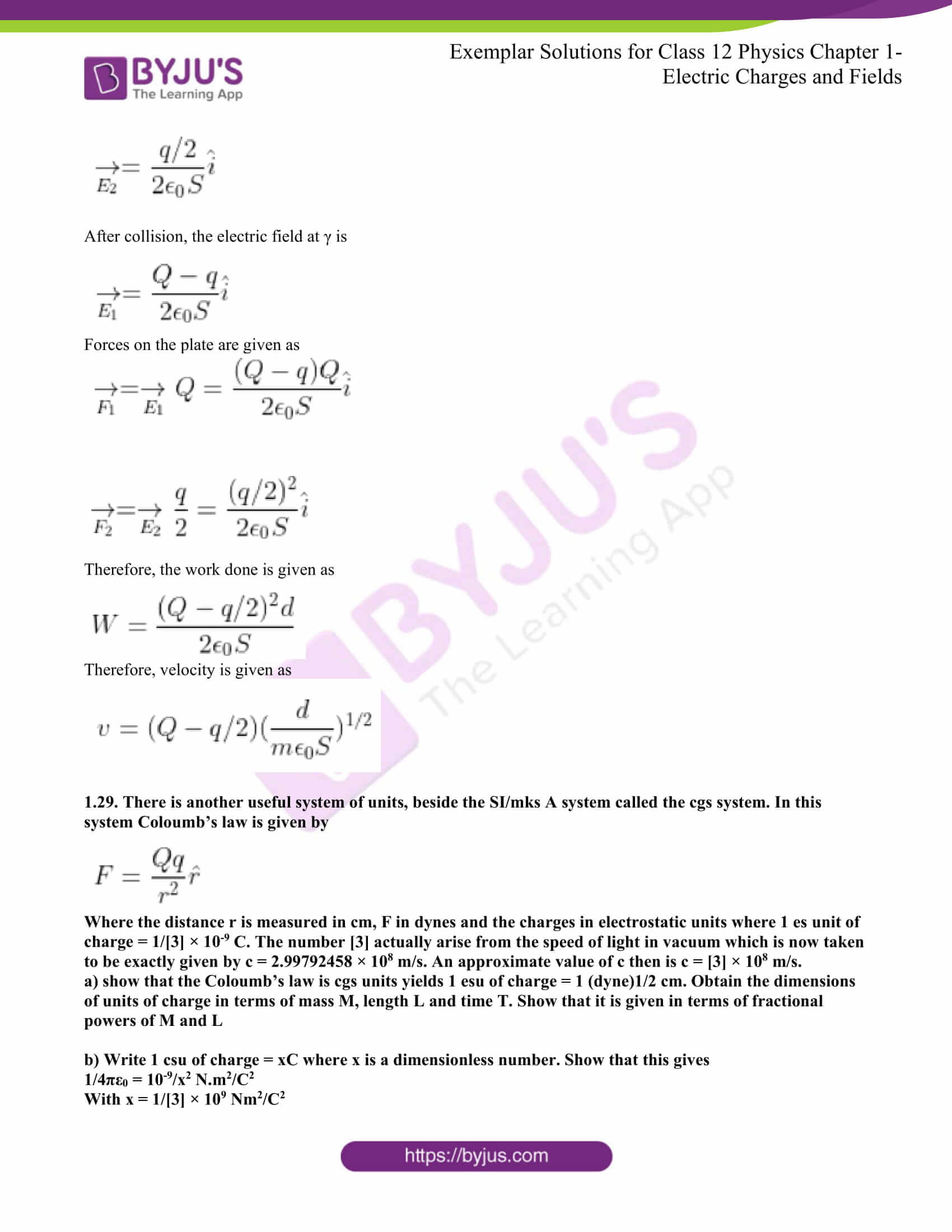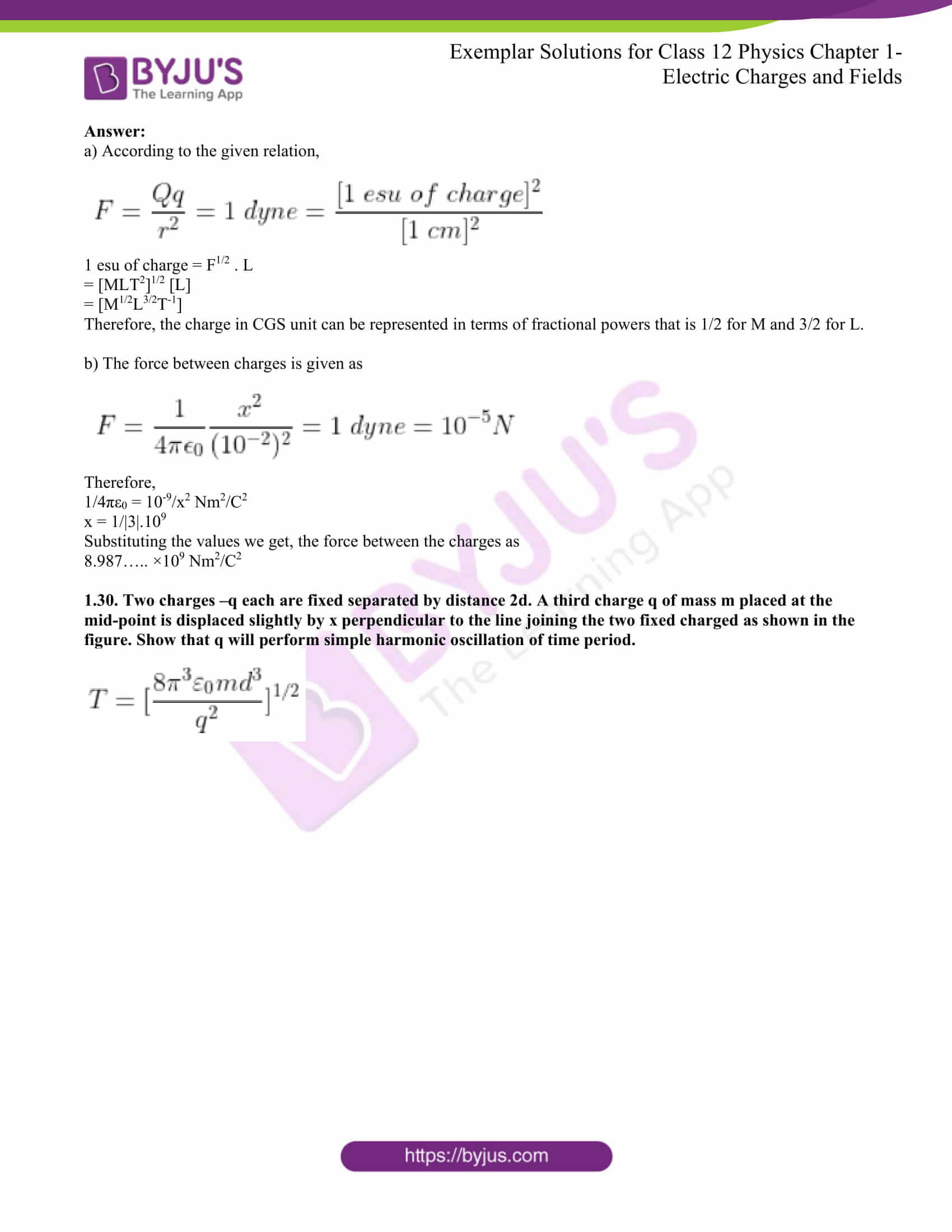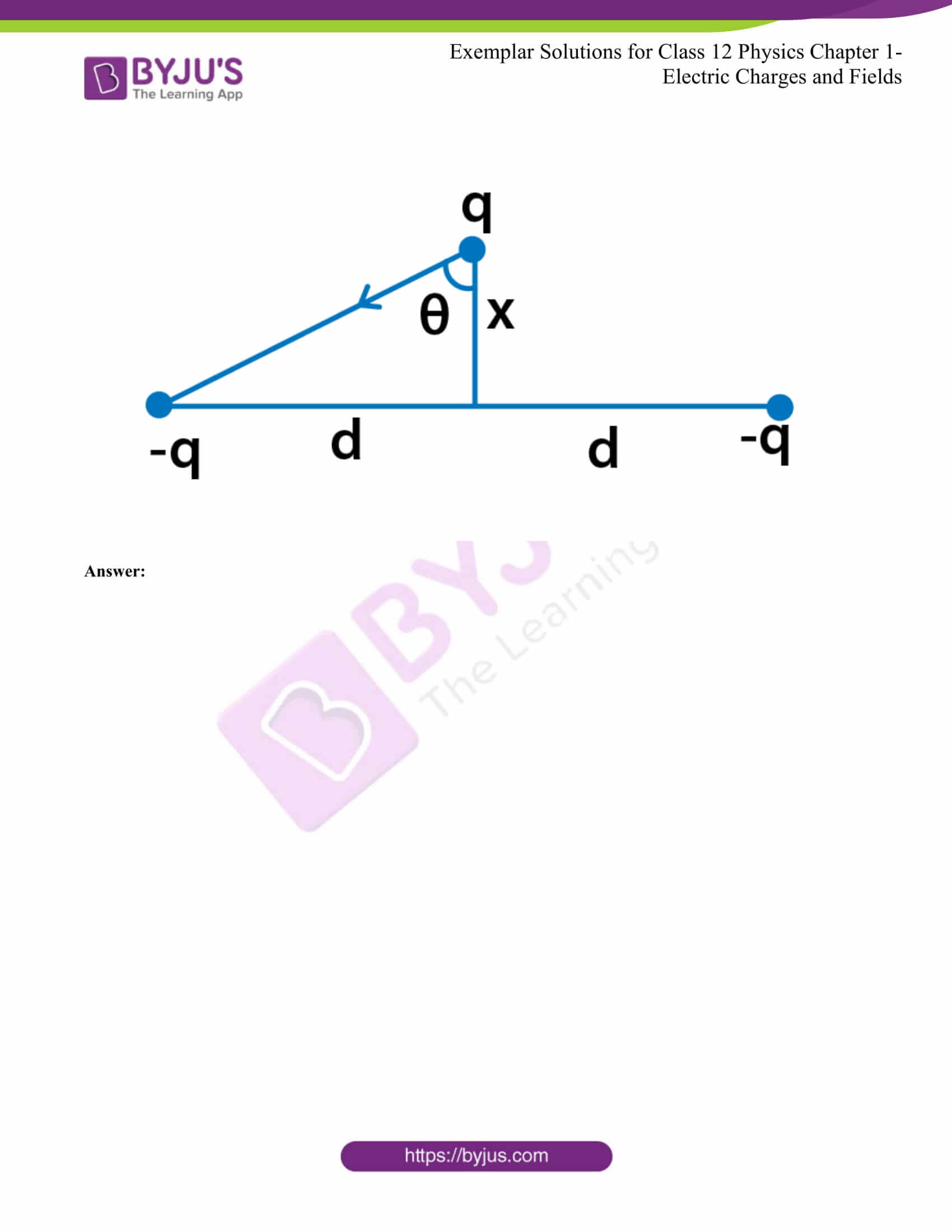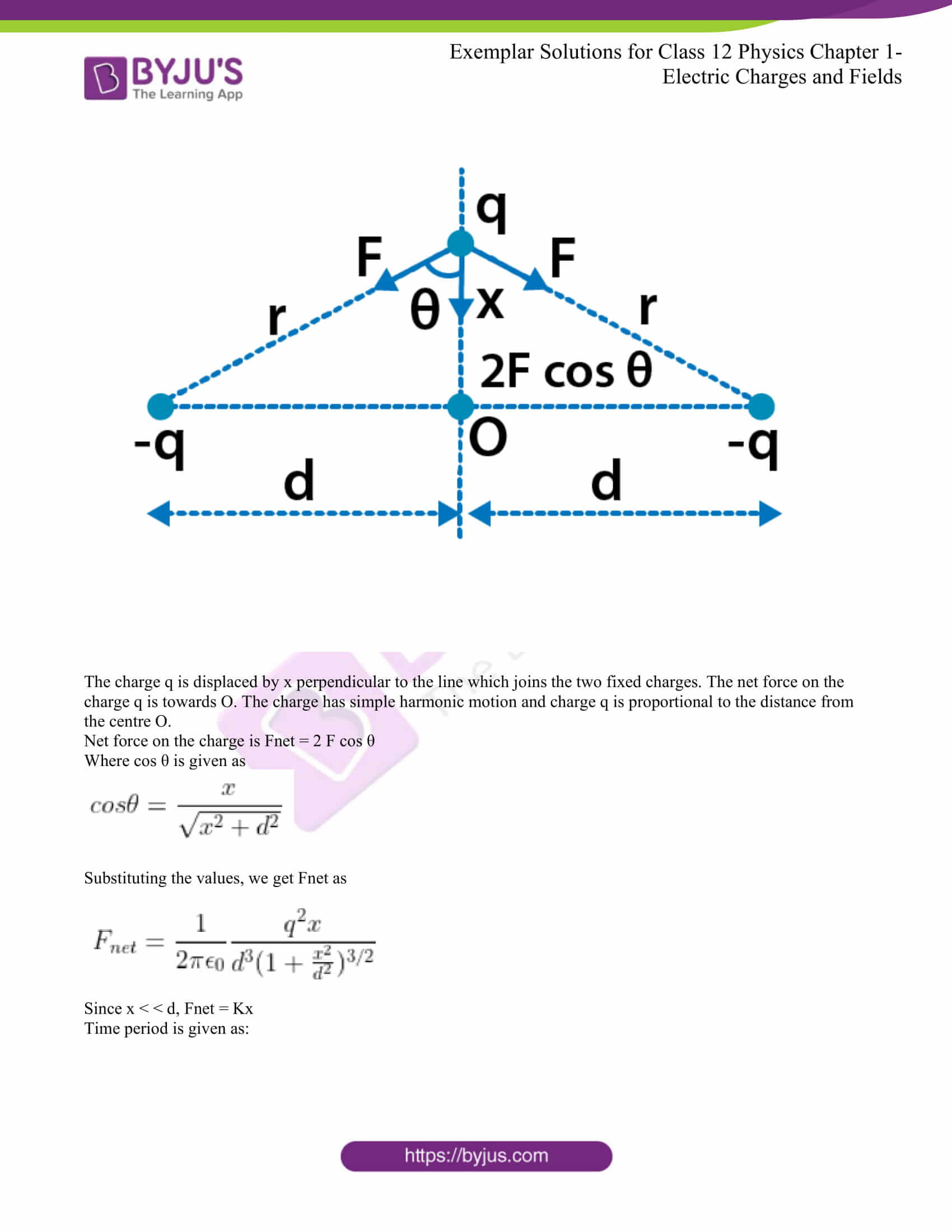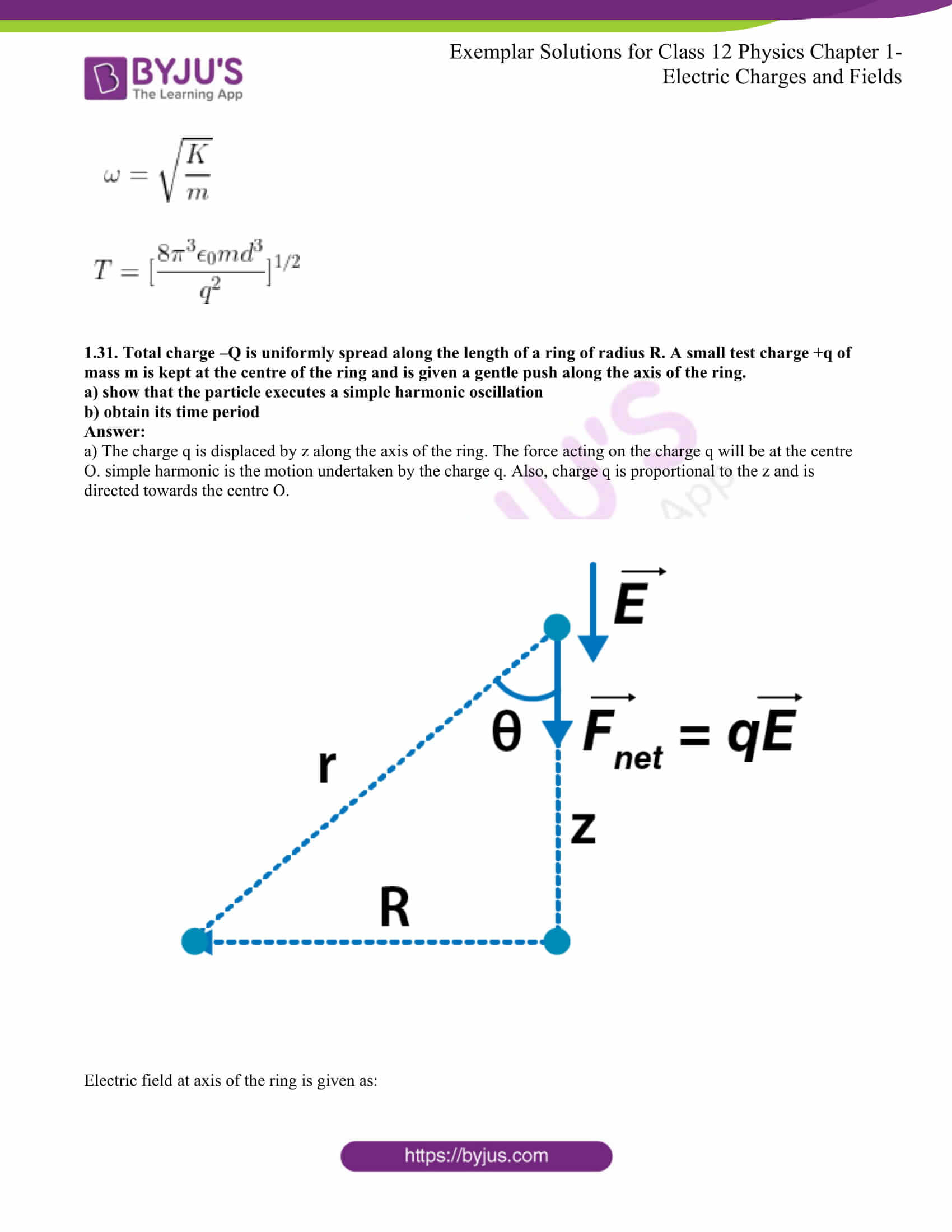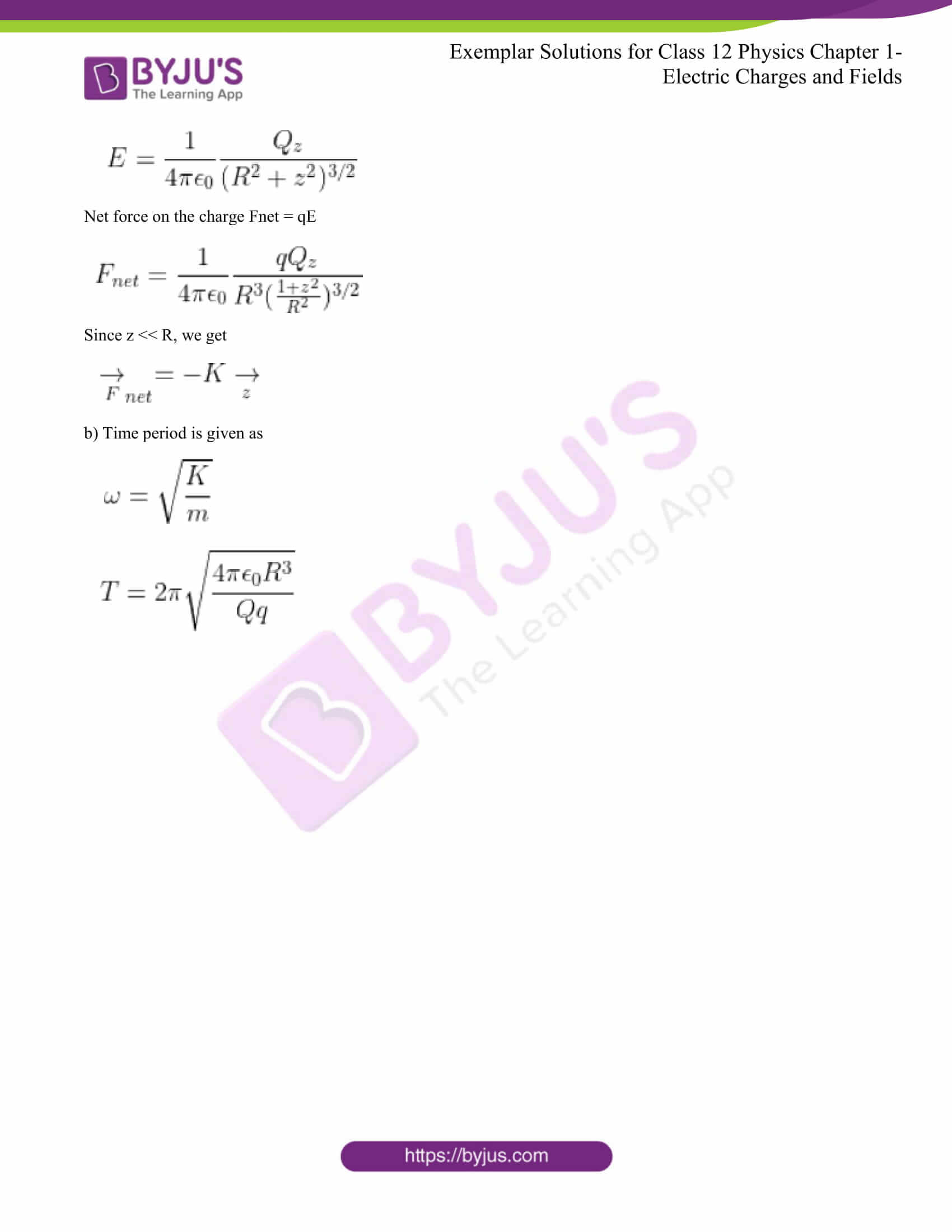### Multiple Choice Questions I

1.1. In the figure, two positive charges q2 and q3 fixed along the y-axis, exert a net electric force in the +x direction on a charge q1 fixed along the x-axis. If a positive charge Q is added at (x,0) the force on q1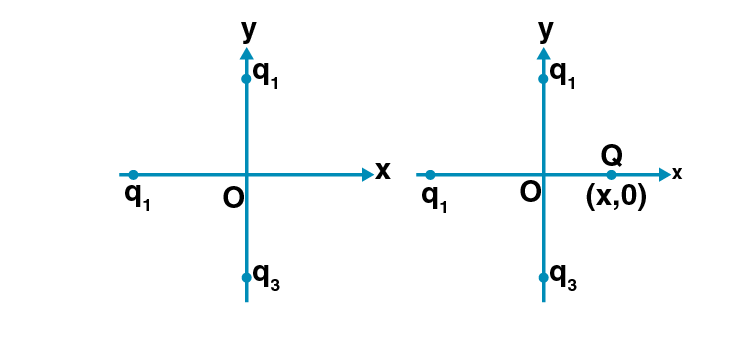a) shall increase along the positive x-axis

b) shall decrease along the positive x-axis

c) shall point along the negative x-axis

d) shall increase but the direction changes because of the intersection of Q with q2 and q3

The correct answer is a) shall increase along the positive x-axis.

1.2. A point positive charge is brought near an isolated conducting sphere. The electric field is best given by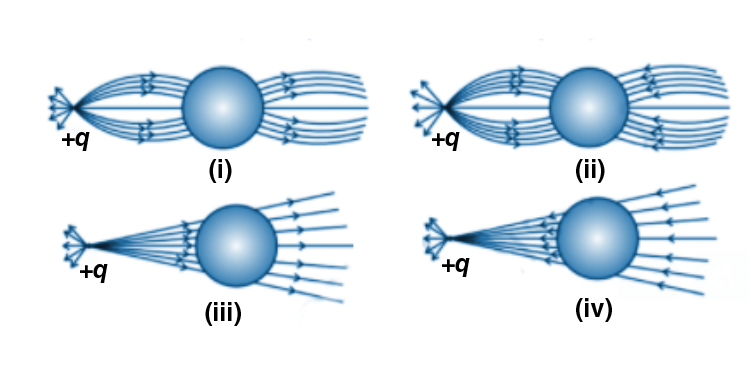a) fig (i)

b) fig (ii)

c) fig (iii)

d) fig (iv)

The correct answer is a) fig (i)

1.3. The electric flux through the surface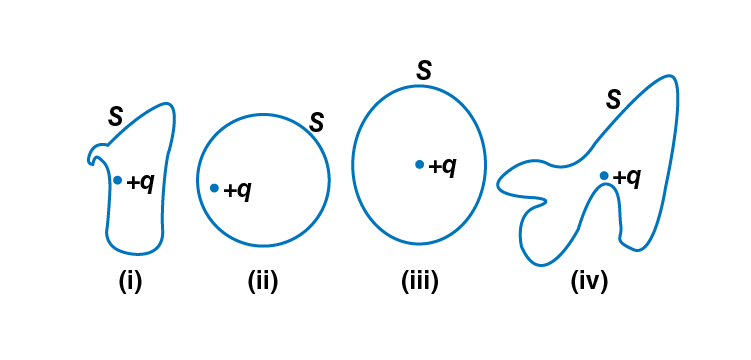a) fig (iv) is the largest

b) fig (iii) is the least

c) fig (ii) is the same as fig (iii) but is smaller than fig (iv)

d) is the same for all the figures

The correct answer is d) is the same for all the figures

1.4. Five charges q1, q2, q3, q4, and q5 are fixed at their positions as shown in the figure. S is a Gaussian surface. The Gauss’s law is given by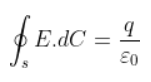Which of the following statements is correct?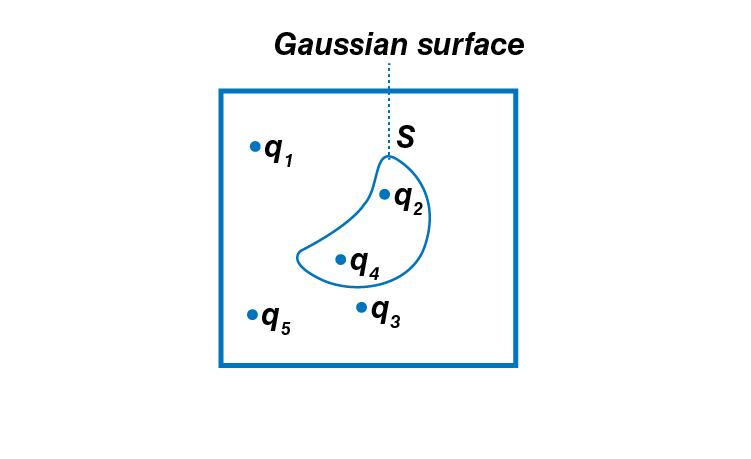a) E on the LHS of the above equation will have a contribution from q1, q3, and q5 while q on the RHS will have a contribution from q2 and q4 only

b) E on the LHS of the above equation will have a contribution from all charges while q on the RHS will have a contribution from q2 and q4 only

c) E on the LHS of the above equation will have a contribution from all charges while q on the RHS will have a contribution from q1, q3, and q5 only

d) both E on the LHS and q on the RHS will have contributions from q2 and q4 only

The correct answer is b) E on the LHS of the above equation will have a contribution from all charges while q on the RHS will have a contribution from q2 and q4 only

1.5. Figure shows electric field lines in which an electric dipole p is placed as shown. Which of the following statements is correct?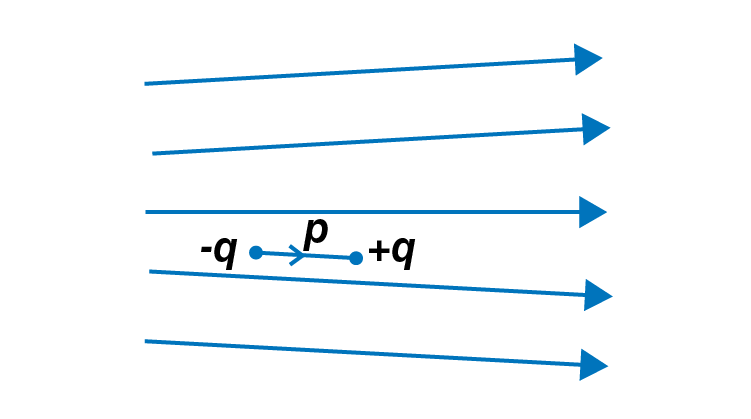a) the dipole will not experience any force

b) the dipole will experience a force towards right

c) the dipole will experience a force towards left

d) the dipole will experience a force upwards

The correct answer is c) the dipole will experience a force towards left

1.6. A point charge +q is placed at a distance d from an isolated conducting plane. The field at a point P on the other side of the plane is

a) directed perpendicular to the plane and away from the plane

b) directed perpendicularly to the plane towards the plane

c) directed radially away from the point charge

d) directed radially towards the point charge

The correct answer is a) directed perpendicular to the plane and away from the plane

1.7. A hemisphere is uniformly charged positively. The electric field at a point on a diameter away from the centre is directed

a) perpendicular to the diameter

b) parallel to the diameter

c) at an angle tilted towards the diameter

d) at an angle tilted away from the diameter

The correct answer is a) perpendicular to the diameter

### Multiple Choice Questions II

1.8. If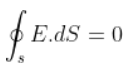over a surface, then

a) the electric field inside the surface and on it is zero

b) the electric field inside the surface is necessarily uniform

c) the number of flux lines entering the surface must be equal to the number of flux lines leaving it

d) all charges must necessarily be outside the surface

c) the number of flux lines entering the surface must be equal to the number of flux lines leaving it

d) all charges must necessarily be outside the surface

1.9. The electric field at a point is

a) always continuous

b) continuous if there is no charge at that point

c) discontinuous only if there is a negative charge at that point

d) discontinuous if there is a charge at that point

b) continuous if there is no charge at that point

d) discontinuous if there is a charge at that point

1.10. If there were only one type of charge in the universe, then

a)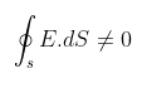on any surface

b)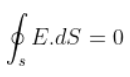if the charge is outside the surface

c)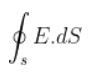could not be defined

d)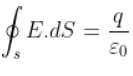if charges of magnitude q were inside the surface

b)if the charge is outside the surface

d)if charges of magnitude q were inside the surface

1.11. Consider a region inside which there are various types of charges but the total charge is zero. At points outside the region

a) the electric field is necessarily zero

b) the electric field is due to the dipole moment of the charge distribution only

c) the dominant electric field is proportional to 1/r3 for large r, where r is the distance from an origin in this region

d) the work done to move a charged particle along a closed path, away from the region, will be zero

c) the dominant electric field is proportional to 1/r3 for large r, where r is the distance from an origin in this region

d) the work done to move a charged particle along a closed path, away from the region, will be zero

1.12. Refer to the arrangement of charges in the figure and a Gaussian surface of radius R with Q at the centre. Then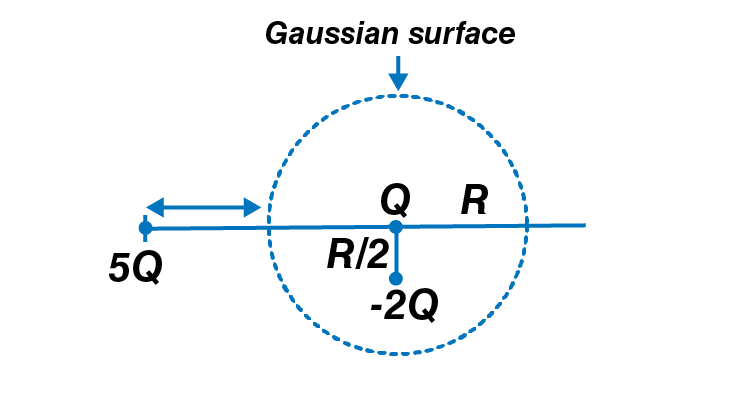a) total flux through the surface of the sphere is –Q/ε0

b) field on the surface of the sphere is –Q/4πε0R2

c) flux through the surface of the sphere due to 5Q is zero

d) field on the surface of the sphere due to -2Q is the same everywhere

a) total flux through the surface of the sphere is –Q/ε0

c) flux through the surface of the sphere due to 5Q is zero

1.13. A positive charge Q is uniformly distributed along a circular ring of radius R. A small test charge q is placed at the centre of the ring. Then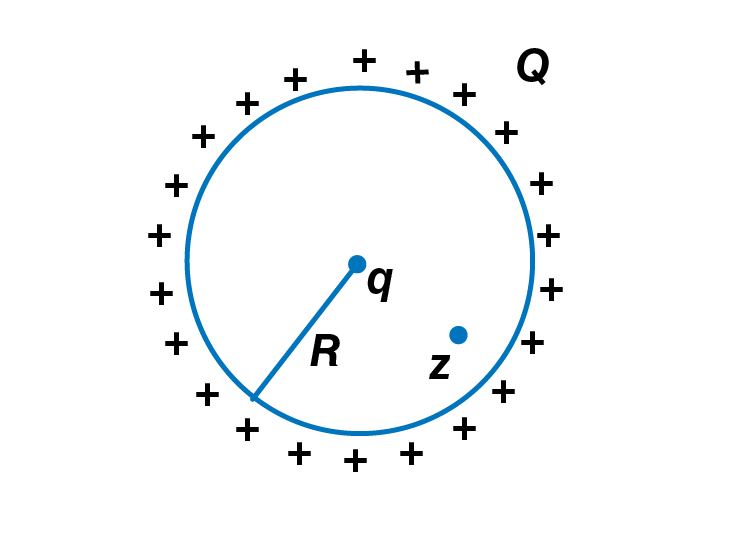a) if q > 0 and is displaced away from the centre in the plane of the ring., it will be pushed back towards the centre

b) if q < 0 and is displaced away from the centre in the plane of the ring, it will never return to the centre and will continue moving till it hits the ring

c) if q < 0, it will perform SHM for small displacement along the axis

d) q at the centre of the ring is in an unstable equilibrium within the plane of the ring for q > 0

a) if q > 0 and is displaced away from the centre in the plane of the ring., it will be pushed back towards the centre

b) if q < 0 and is displaced away from the centre in the plane of the ring, it will never return to the centre and will continue moving till it hits the ring

d) q at the centre of the ring is in an unstable equilibrium within the plane of the ring for q > 0

1.14. An arbitrary surface encloses a dipole. What is the electric flux through this surface?

According to Gauss law, for an enclosed surface, the electric flux is given as: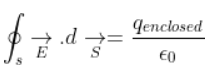The net charge on the dipole is given as –q and +q whose sum is equal to zero.

1.15. A metallic spherical shell has an inner radius R1 and outer radius R2. A charge Q is placed at the centre of the spherical cavity. What will be surface charge density on

a) the inner surface

b) the outer surface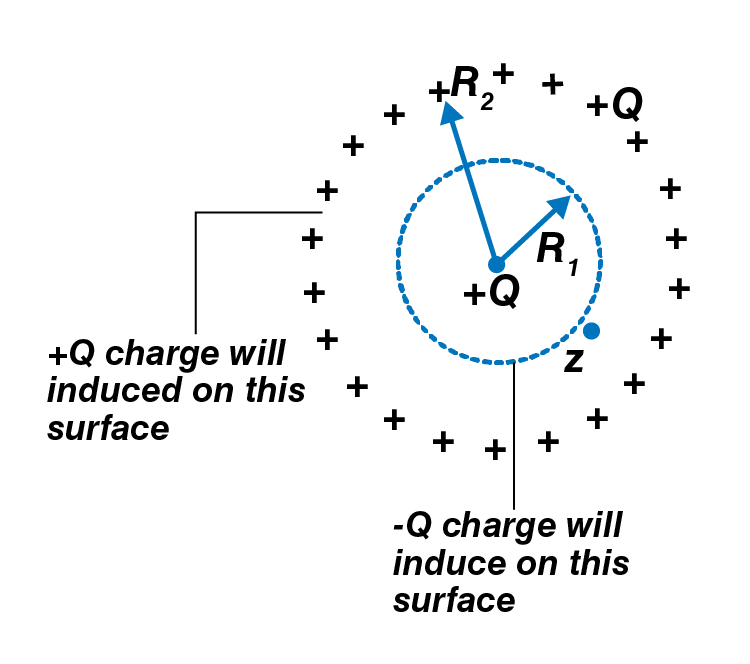Q is the charge which is kept at the centre of the spherical cavity. –Q is the charge that is induced in the inner surface and –Q on the outer surface.

σ is the surface charge density

a) σ1 is the surface charge density on the inner surface = Charge/Area =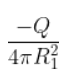b) σ2 is the surface charge density on the outer surface =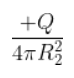1.16. The dimensions of an atom are of the order of an Angstrom. Thus there must be large electric fields between the protons and electrons. Why, then is the electrostatic field inside a conductor zero?

The electrostatic field inside a conductor is zero as the dimensions of the atom are in the order of an Angstrom and the electrostatic field inside the conductor is caused by the presence of the excess charges.

1.17. If the total charge enclosed by a surface is zero, does it imply that the electric field everywhere on the surface is zero? Conversely, if the electric field everywhere on a surface is zero, does it imply that net charge inside is zero.

The total charge enclosed by a surface is zero, it doesn’t imply that the electric field everywhere on the surface is zero and the field may be normal to the surface. Also, the conversely it does imply that the electric field everywhere on the surface is zero.

1.18. Sketch the electric field lines for a uniformly charged hollow cylinder shown in the figure.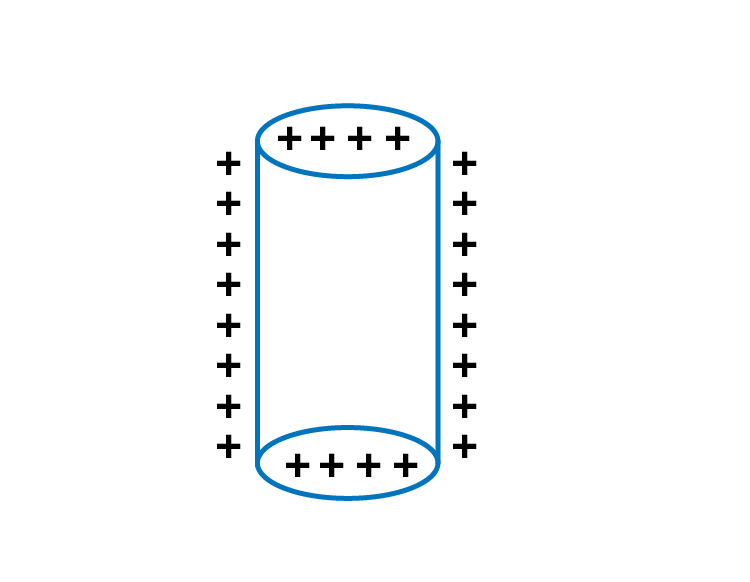Following are the side view and top view of the electric field lines for a uniformly charged hollow cylinder.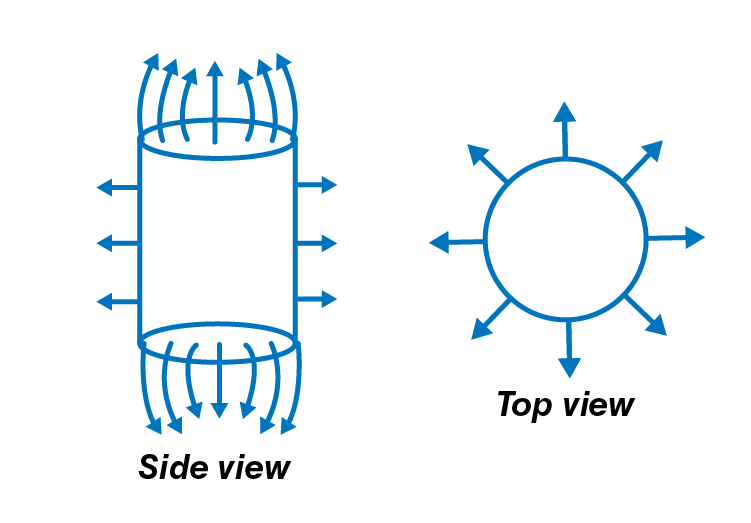1.19. What will be the total flux through the faces of the cube with a side of length a if a charge q is placed at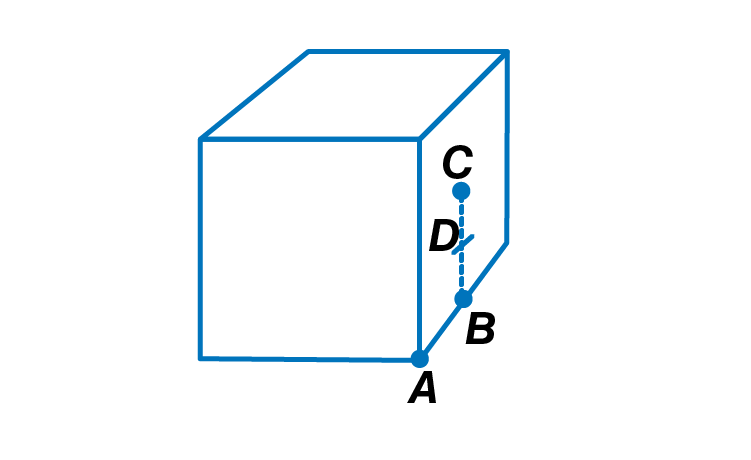a) A: a corner of the cube

b) B: mid-point of an edge of the cube

c) C: centre of a face of the cube

d) D: mid-point of B and C

a) When we consider the charged particle to be placed at the centre of the cube whose side is 2a, then the charge is equally distributed among 8 cubes. Therefore, the total flux through the faces of the cube = q/8ε0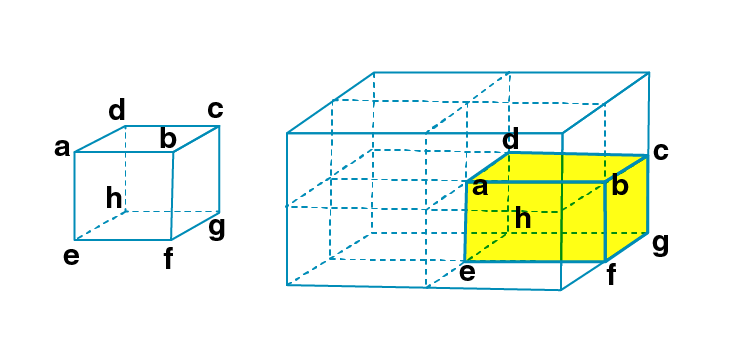b) When the charge is placed at B, the charge is equally distributed among the 4 cubes. Therefore, the total flux through the four faces is given as = q/4ε0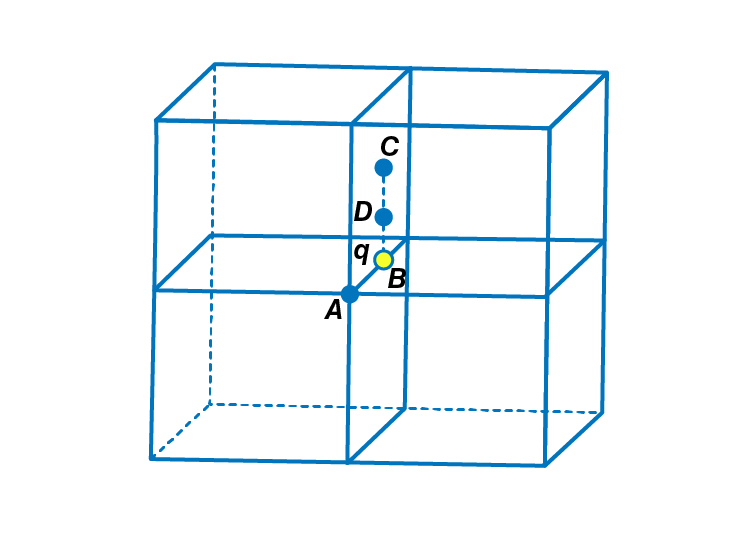c) When the charge is placed at C, the charge is shared among 2 cubes equally. Therefore, the total flux through these faces is given as = q/2ε0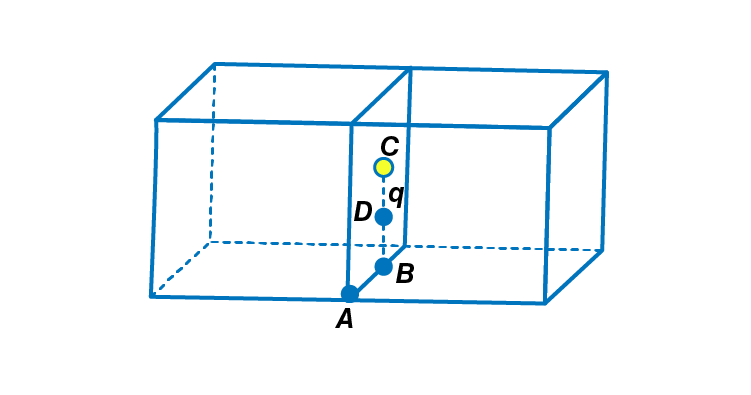d) When the charge is placed at D, the charge is distributed among two cubes and therefore, the total flux is given as = q/2ε0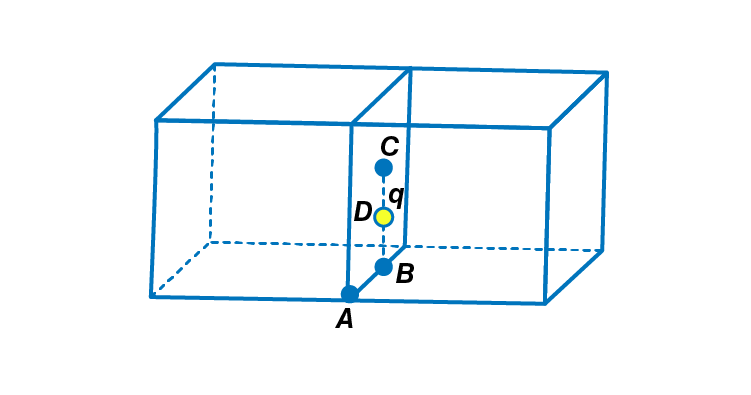1.20. A paisa coin is made up of Al-Mg alloy and weighs 0.75 g. It has a square shape and its diagonal measures 17 mm. It is electrically neutral and contains equal amounts of positive and negative charges. Treating the paisa coins made up of only Al, find the magnitude of an equal number of positive and negative charges. What conclusion do you draw from this magnitude?

1 molar mass M of Al = 6.023 × 1023 atoms

No.of aluminium atoms in one paise coin = N = 6.023 × 1023/26.9815 × 0.75 = 1.76 ×1022

There are 13 protons and 13 electrons on the Al as the charge number is 13

Magnitude of positive and negative charges = 3.48 × 104 C = 34.8 kC

1.21. Consider a coin of example 1.20. It is electrically neutral and contains equal amounts of positive and negative charge of magnitude 34.8 kC. Suppose that these equal charges were concentrated in two-point charges separated by

a) 1 cm

b) 100 m

Find the force on each such point charge in each of the three cases. What do you conclude from these results?

a) F1 = |q|2/4πε0r12 = 1.09 × 1023 N

b) F2 = |q|2/4πε0r22 = 1.09 × 1015 N

c) F3 = |q|2/4πε0r32 = 1/09 × 107 N

1.22. Figure represents a crystal unit of caesium chloride, CsCl. The caesium atoms, represented by open circles are situated at the corners of a cube of side 0.40 nm, whereas a Cl atom is situated at the centre of the cube. The Cs atoms are deficient in one electron while the Cl atom carries an excess electron

a) what is the net electric field on the Cl atom due to eight Cs atoms?

b) suppose that the Cs atom at the corner A is missing. What is the net force now on the Cl atom due to seven remaining Cs atoms?

a) The net electric field at the centre of the cube is zero as the chlorine atom is distributed equally from all the eight corners of the cube from the centre.

b) The vector sum of the electric field due to charge A is EA + Eseven charges = 0

1.23. Two charges q and -3q are placed fixed on x-axis separated by distance ‘d’. Where should a third charge 2q be placed such that it will not experience any force?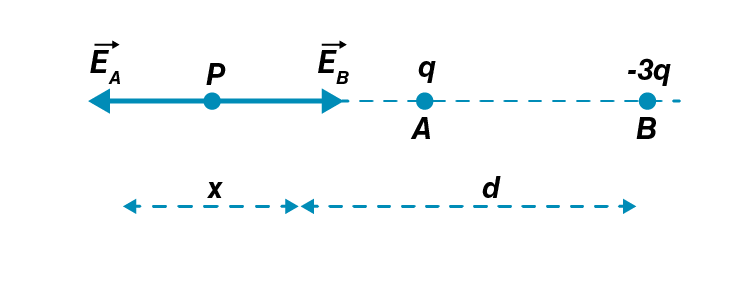At point P,

q/4πε0 x2 = 3q/4πε0 (x+d)2

(x + d)2 = 3x2

Solving the above equation, we get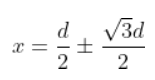Therefore, the distance between the 2q and q charges is d/2(1+√3)

1.24. Figure shows the electric field lines around three-point charges A, B, and C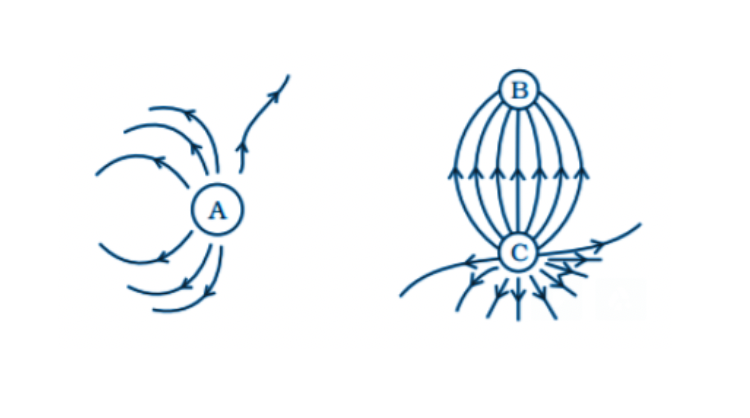a) which charges are positive?

b) which charge has the largest magnitude? Why?

c) in which region or regions of the picture could the electric field be zero?

i) near A

ii) near B

iii) near C

iv) nowhere

a) A and C are positive as electric lines come out from the positive charge.

b) The magnitude of C is maximum than A and B because as the electric lines of forces from charge increases, the intensity of the electric field increases.

c) The electric field will be zero between A and C as that is the neutral point.

1.25. Five charges, q each are placed at the corners of a regular pentagon of side ‘a’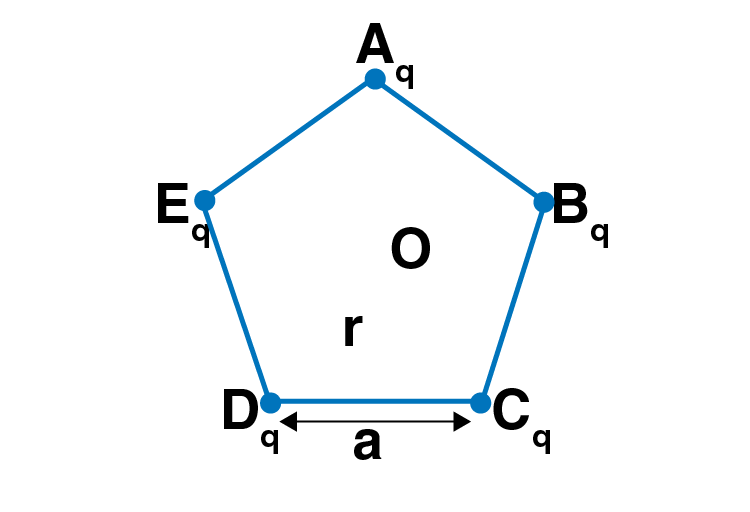a) i) what will be the electric field at O, the centre of the pentagon?

ii) what will be the electric field at O if the charge from one of the corners is removed?

iii) what will be the electric field at O if the charge q at A is replaced by –q?

b) how would you answer to a) be affected if pentagon is replaced by an n-sided regular polygon with charge q at each of its corners?

a) i) The net electric field at O will be zero as it is symmetric to all the five charges.

ii) Following is the electric field at O if the charge from one of the corners is removed:

E1 = -1q/4πε0r2

Also, the direction of the electric field will be from O to A.

iii) Following is the electric field at O if the charge q at A is replaced by –q:

E = -2q/4πε0r2

b) When an n-sided regular polygon with charge q is replaced at each of the corners, then

i) Again at centre O, the net electric field will be zero.

ii) The direction of the electric field will be from O to opposite of OA and the electric field is given as:

E2 = -q/4πε0r2

iii) The electric field will be from O to A and is given as:

E = -2q/4πε0r2

1.26. In 1959 Lyttleton and Bondi suggested that the expansion of the universe could be explained if matter carried a net charge. Suppose that the universe is made up of hydrogen atoms with a number density N, which is maintained a constant. Let the charge on the proton be: ep = -(1 + y) e where e is the electronic charge.

a) find the critical value of y such that expansion may start

b) show that the velocity of expansion is proportional to the distance from the centre

a) There is one proton and one electron charge on each hydrogen atom.

eH = eP + e = -(1+y)e+e=-ye=|ye|

E is the electric field intensity at a distance R on the surface and according to Gauss theorem,

E = 1/3 N|ye|R/ε0

Solving for y we get, y = 10-18

b) Ne force experienced by the hydrogen atom is F = Fc – FG

The acceleration experienced by the hydrogen atom is given as d2R/dt2 = α2R

v = dR/dt = αR

Therefore, v is proportional to R that velocity is proportional to the distance from the centre.

1.27. Consider a sphere of radius R with charge density distributed as

ρ(r) = kr for r ≤ R

ρ(r) = 0 for r > R

a) find the electric field at all points r

b) suppose the total charge on the sphere is 2e where e is the electron charge. Where can two protons be embedded such that the force on each of them is zero? Assume that the introduction of the proton does not alter the negative charge distribution

a)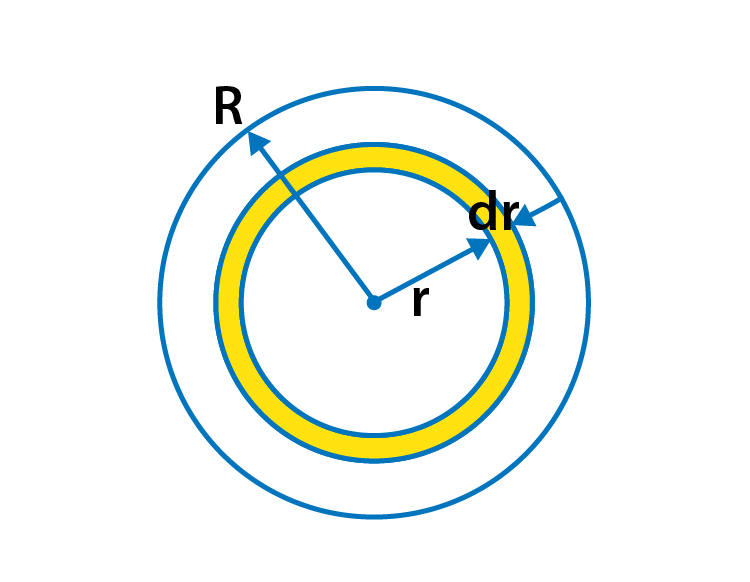S is the sphere with R as the radius. In the above figure, there are two hypothetic spheres of radius r < R and r > R.

When the r < R, the electric field intensity is given as:

E = 1/4ε0 Kr2

When the r > R, the electric field intensity is given as:

E = K/4ε0 R4/r2

b)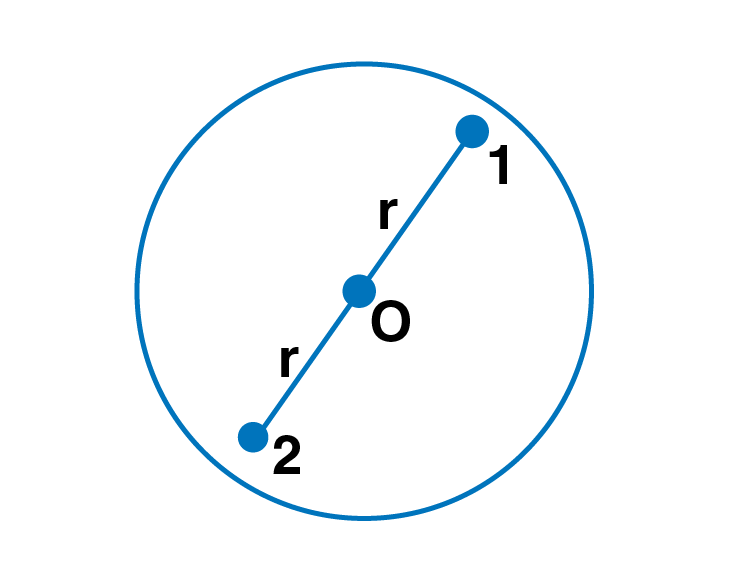The two protons are kept symmetrically opposite to each other along the diameter. The charge on the sphere is given as:

K = 2e/πR4

The attractive forces between the proton 1 and proton 2 are given as:

F1 = eE = -eKr2/4ε0

F2 = e2/4πε0 (2r)2

Using the net force on the proton, we can calculate the distance between the protons, ‘r’ as follows

r = R/(8)1/4

1.28. Two fixed, identical conducting plates (α and β), each of surface area S are charged to –Q and q respectively, where Q > q > 0. A third identical plate (γ), free to move is located on the other side of the plate with charge q at a distance d. The third plate is released and collides with the plate β. Assume the collision is elastic and the time of collision is sufficient to redistributed charge among β and γ.

a) find the electric field acting on the plate γ before collision

b) find the charges on β and γ after the collision

c) find the velocity of the plate γ after the collision and at a distance d from the plate β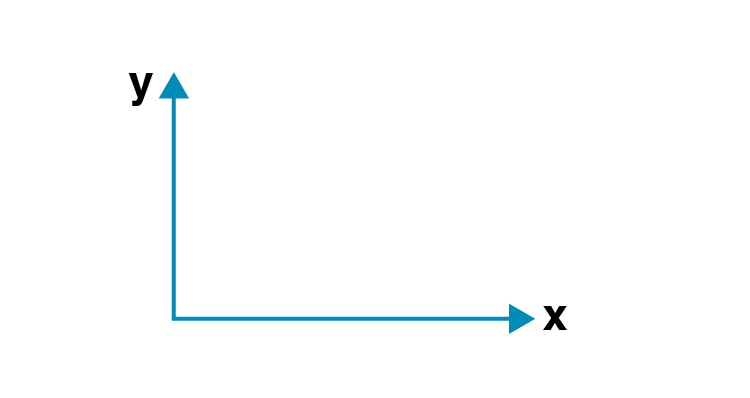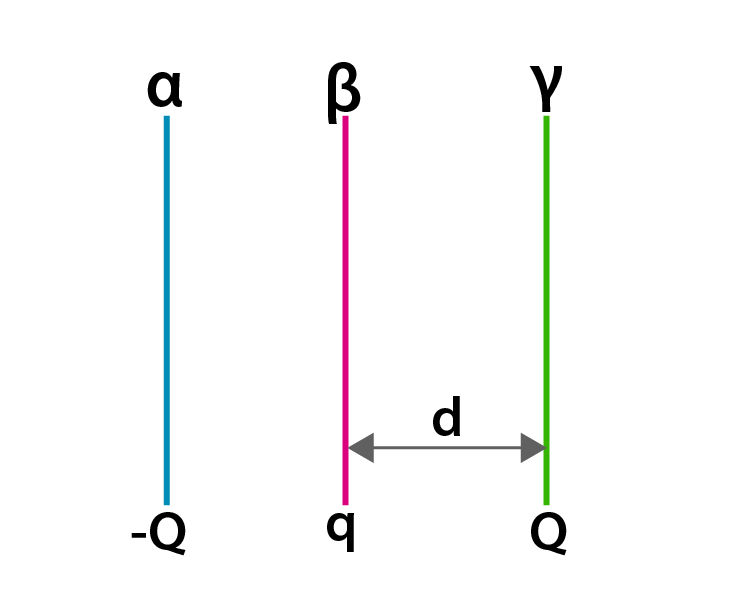a) The electric field at plate γ due to plate α isThe electric field at plate γ due to plate β is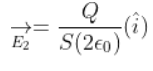Adding both the equations, we get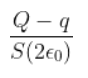if Q > q

b) Electric field at O due to plate α,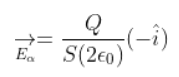Electric field at O due to plate β,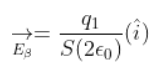Electric field at O due to plate γ,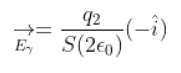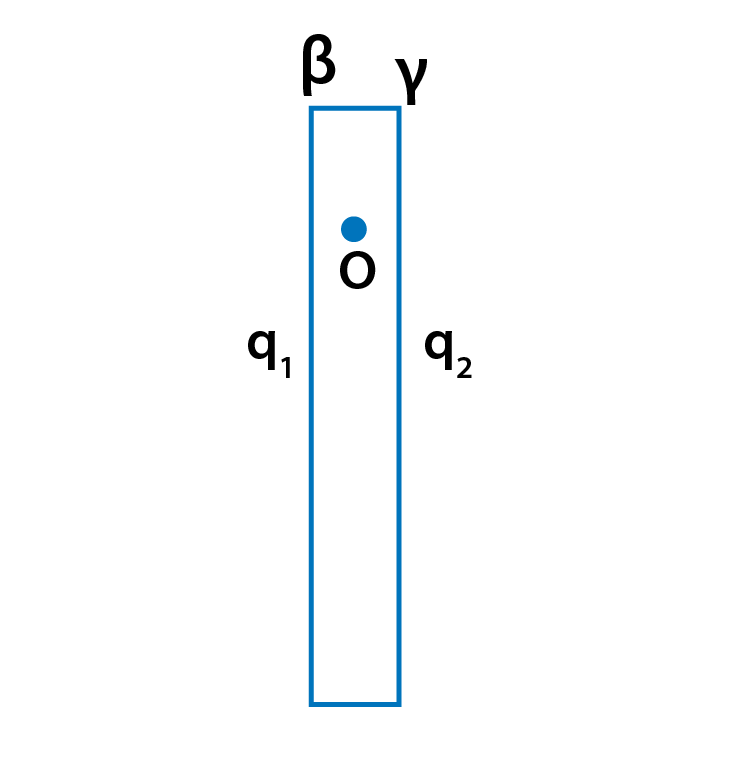Solving the equations, we get

q1 = (Q + q/2) = charge on plate β

q1 = (q/2) = charge on plate γ

c) Let v be the velocity and d be the distance from plate β after collision.

The mass on the plate γ is m and the KE is equal to the work done by the electric field.

Following is the equation after collision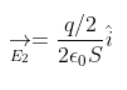After collision, the electric field at γ is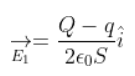Forces on the plate are given as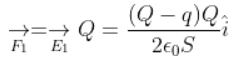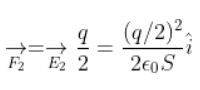Therefore, the work done is given as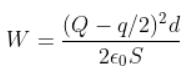Therefore, velocity is given as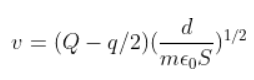1.29. There is another useful system of units, besides the SI/mks A system called the cgs system. In this system Columb’s law is given by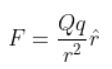Where the distance r is measured in cm, F in dynes and the charges in electrostatic units where 1 es unit of charge = 1/ × 10-9 C. The number  actually arise from the speed of light in vacuum which is now taken to be exactly given by c = 2.99792458 × 108 m/s. An approximate value of c then is c =  × 108 m/s.

a) show that the Columb’s law is cgs units yields 1 esu of charge = 1 (dyne)1/2 cm. Obtain the dimensions of units of charge in terms of mass M, length L and time T. Show that it is given in terms of fractional powers of M and L

b) Write 1 csu of charge = xC where x is a dimensionless number. Show that this gives

1/4πε0 = 10-9/x2 N.m2/C2

With x = 1/ × 109 Nm2/C2

a) According to the given relation,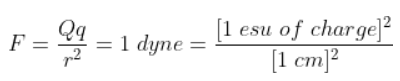1 esu of charge = F1/2 . L

= [MLT2]1/2 [L]

= [M1/2L3/2T-1]

Therefore, the charge in the CGS unit can be represented in terms of fractional powers that is 1/2 for M and 3/2 for L.

b) The force between charges is given as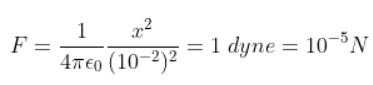Therefore,

1/4πε0 = 10-9/x2 Nm2/C2

x = 1/|3|.109

Substituting the values we get, the force between the charges as

8.987….. ×109 Nm2/C2

1.30. Two charges –q each are fixed separated by distance 2d. A third charge q of mass m placed at the mid-point is displaced slightly by x perpendicular to the line joining the two fixed charged as shown in the figure. Show that q will perform simple harmonic oscillation of time period.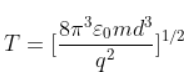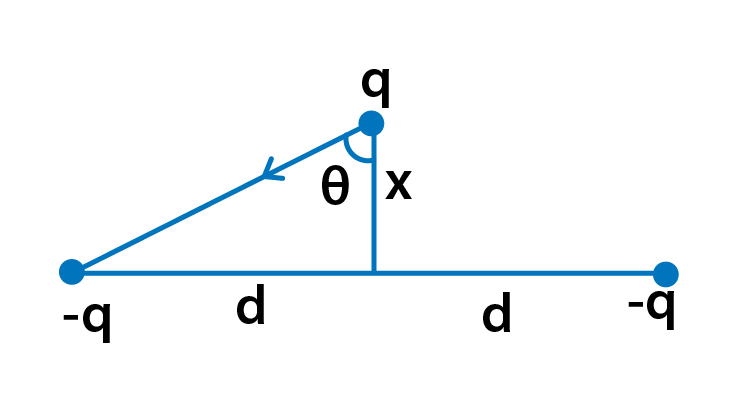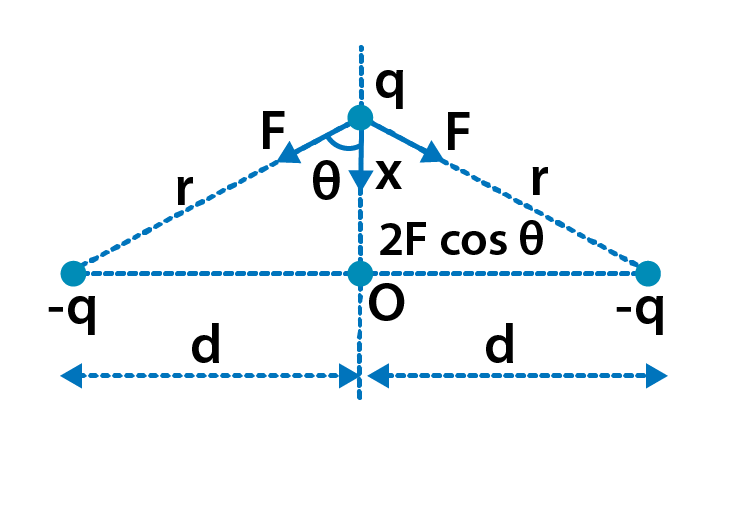The charge q is displaced by x perpendicular to the line which joins the two fixed charges. The net force on the charge q is towards O. The charge has simple harmonic motion and charge q is proportional to the distance from the centre O.

The net force on the charge is Fnet = 2 F cos θ

Where cos θ is given as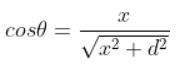Substituting the values, we get Fnet as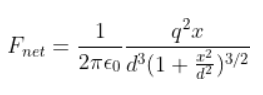Since x < < d, Fnet = Kx

Time period is given as: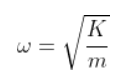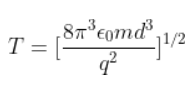1.31. Total charge –Q is uniformly spread along the length of a ring of radius R. A small test charge +q of mass m is kept at the centre of the ring and is given a gentle push along the axis of the ring.

a) show that the particle executes a simple harmonic oscillation

b) obtain its time period

a) The charge q is displaced by z along the axis of the ring. The force acting on the charge q will be at the centre O. simple harmonic is the motion undertaken by the charge q. Also, charge q is proportional to the z and is directed towards the centre O.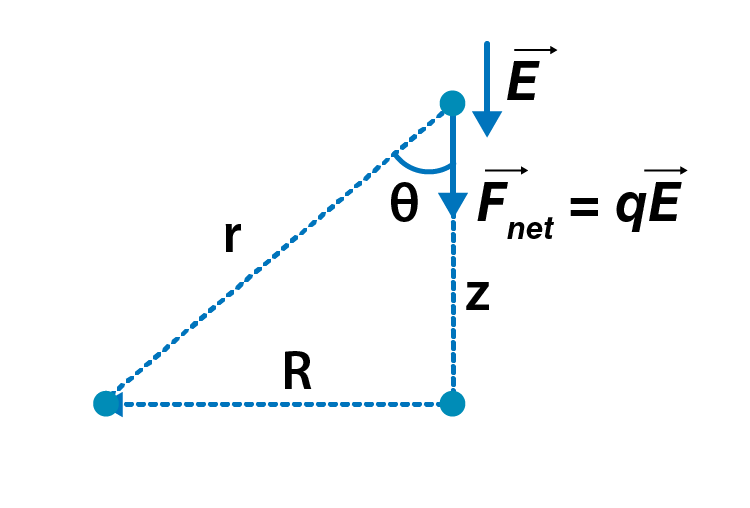Electric field at the axis of the ring is given as: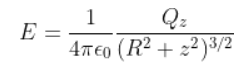The net force on the charge Fnet = qE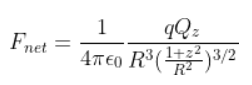Since z << R, we get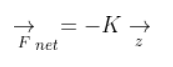b) Time period is given as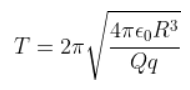### NCERT Exemplar Problems Class 12 Physics – Electric Charges and Fields

When matter experiences a force when placed in a magnetic field, the property of matter is called an electric charge. The field surrounding an electric charge is called an electromagnetic field, where opposite charges attract each other. It is a scalar quantity, and the charge can be either positive or negative in nature. Electric charges are measured in coulombs, and they can be quantised in nature.

### The Important Concepts of Electric Charges and Fields NCERT Exemplar Solutions

1. Electric Charge – Introduction to charges, historical experiments, detection of charge, the electroscope and other experiments
2. Conductors and Insulators – Definitions, electric circuits and properties
3. Charging by Induction – Pith and ball experiment, charge by induction and metal sphere experiment
4. Basic Properties of Electric Charge
2. Charge is conserved
3. Quantisation of charge
5. Coulomb’s Law – Derivation, formulas, application and numerical problems
6. Forces between Multiple Charges – Calculation of force, formula derivation and calculations
7. Electric Field – Calculation, formula derivation and applications
1. Electric field due to a system of charges
2. The physical significance of the electric field
8. Electric Field Lines – Meaning, pictorial representation and applications
9. Electric Flux -Definition, formula derivation and calculations – Derivation of Torque, applications and calculations
10. Electric Dipole
1. The field of an electric dipole
2. The physical significance of dipoles
3. Dipole in a uniform external field
11. Continuous Charge Distribution – Derivation of surface charge density, linear charge density and volume charge density, derivation of formulas and calculation of problems
12. Gauss’s Law – Statement, derivation and calculations
13. Applications of Gauss’s Law
14. Field due to an infinitely long straight, uniformly charged wire
1. Field due to a uniformly charged infinite plane sheet
2. Field due to a uniformly charged thin spherical shell

Technology has revolutionised education, and  BYJU’S is using technology to transform learning. BYJU’S innovative approach helps students learn the concepts thoroughly. Our study materials, NCERT Solutions, previous years’ question papers, sample papers, Physics Exemplar Class 12 chapters, and video and animation lessons aid students in learning the concepts in the NCERT syllabus.